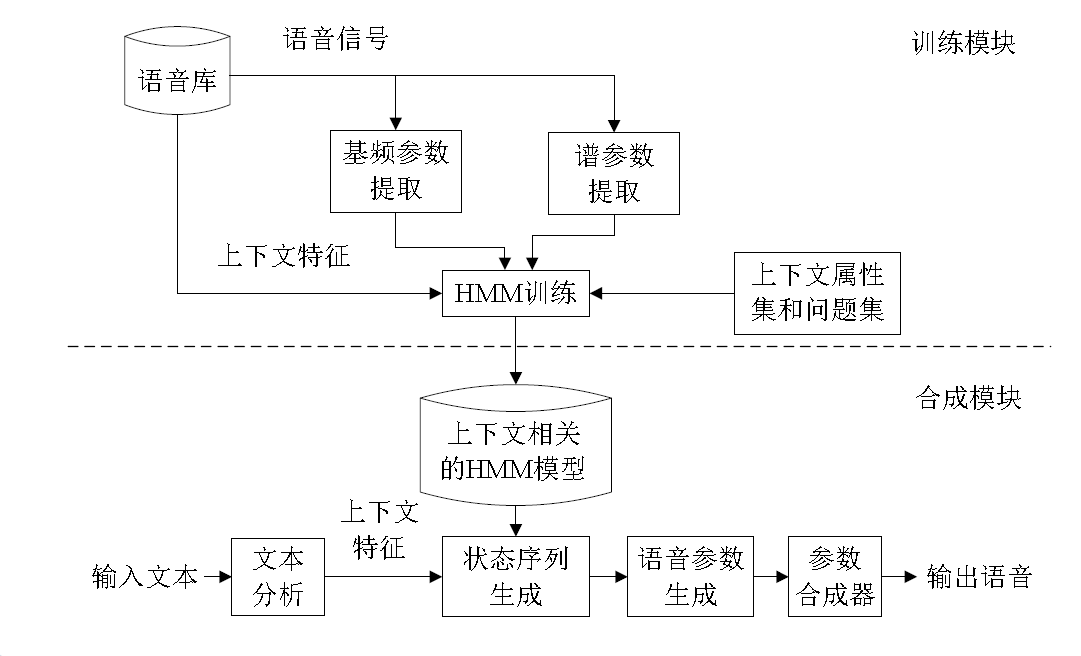2017-04-03 20:28:52 u013131595 阅读数 2375
• ###### C++语音识别开篇

本篇mark老师将教大家使用第三方库的调用来简单的实现语音识别。随着机器学习和人工智能的热闹，国内语音行业也可谓是百花齐放。 语音识别一个伟大的时代已在我们身边悄悄走来。

6113 人正在学习 去看看 杨波

# 1.RNN简介# 2.LSTM简介# 3.BiRNN简介# 4.代码分析

def BiRNN(conf_path, batch_x, seq_length, n_input, n_context):
parser = ConfigParser(os.environ)

dropout = [float(x) for x in parser.get('birnn', 'dropout_rates').split(',')]
relu_clip = parser.getint('birnn', 'relu_clip')

b1_stddev = parser.getfloat('birnn', 'b1_stddev')
h1_stddev = parser.getfloat('birnn', 'h1_stddev')
b2_stddev = parser.getfloat('birnn', 'b2_stddev')
h2_stddev = parser.getfloat('birnn', 'h2_stddev')
b3_stddev = parser.getfloat('birnn', 'b3_stddev')
h3_stddev = parser.getfloat('birnn', 'h3_stddev')
b5_stddev = parser.getfloat('birnn', 'b5_stddev')
h5_stddev = parser.getfloat('birnn', 'h5_stddev')
b6_stddev = parser.getfloat('birnn', 'b6_stddev')
h6_stddev = parser.getfloat('birnn', 'h6_stddev')

n_hidden_1 = parser.getint('birnn', 'n_hidden_1')
n_hidden_2 = parser.getint('birnn', 'n_hidden_2')
n_hidden_5 = parser.getint('birnn', 'n_hidden_5')
n_cell_dim = parser.getint('birnn', 'n_cell_dim')

n_hidden_3 = int(eval(parser.get('birnn', 'n_hidden_3')))
n_hidden_6 = parser.getint('birnn', 'n_hidden_6')

# Input shape: [batch_size, n_steps, n_input + 2*n_input*n_context]
batch_x_shape = tf.shape(batch_x)

# Reshaping batch_x to a tensor with shape [n_steps*batch_size, n_input + 2*n_input*n_context].
# This is done to prepare the batch for input into the first layer which expects a tensor of rank 2.

# Permute n_steps and batch_size
batch_x = tf.transpose(batch_x, [1, 0, 2])
# Reshape to prepare input for first layer
batch_x = tf.reshape(batch_x,
[-1, n_input + 2 * n_input * n_context])  # (n_steps*batch_size, n_input + 2*n_input*n_context)

# The next three blocks will pass batch_x through three hidden layers with
# clipped RELU activation and dropout.

# 1st layer
with tf.name_scope('fc1'):
b1 = variable_on_cpu('b1', [n_hidden_1], tf.random_normal_initializer(stddev=b1_stddev))
h1 = variable_on_cpu('h1', [n_input + 2 * n_input * n_context, n_hidden_1],
tf.random_normal_initializer(stddev=h1_stddev))
layer_1 = tf.minimum(tf.nn.relu(tf.add(tf.matmul(batch_x, h1), b1)), relu_clip)
layer_1 = tf.nn.dropout(layer_1, (1.0 - dropout))

tf.summary.histogram("weights", h1)
tf.summary.histogram("biases", b1)
tf.summary.histogram("activations", layer_1)

# 2nd layer
with tf.name_scope('fc2'):
b2 = variable_on_cpu('b2', [n_hidden_2], tf.random_normal_initializer(stddev=b2_stddev))
h2 = variable_on_cpu('h2', [n_hidden_1, n_hidden_2], tf.random_normal_initializer(stddev=h2_stddev))
layer_2 = tf.minimum(tf.nn.relu(tf.add(tf.matmul(layer_1, h2), b2)), relu_clip)
layer_2 = tf.nn.dropout(layer_2, (1.0 - dropout))

tf.summary.histogram("weights", h2)
tf.summary.histogram("biases", b2)
tf.summary.histogram("activations", layer_2)

# 3rd layer
with tf.name_scope('fc3'):
b3 = variable_on_cpu('b3', [n_hidden_3], tf.random_normal_initializer(stddev=b3_stddev))
h3 = variable_on_cpu('h3', [n_hidden_2, n_hidden_3], tf.random_normal_initializer(stddev=h3_stddev))
layer_3 = tf.minimum(tf.nn.relu(tf.add(tf.matmul(layer_2, h3), b3)), relu_clip)
layer_3 = tf.nn.dropout(layer_3, (1.0 - dropout))

tf.summary.histogram("weights", h3)
tf.summary.histogram("biases", b3)
tf.summary.histogram("activations", layer_3)

# Create the forward and backward LSTM units. Inputs have length n_cell_dim.
# LSTM forget gate bias initialized at 1.0 (default), meaning less forgetting
# at the beginning of training (remembers more previous info)
with tf.name_scope('lstm'):
# Forward direction cell:
lstm_fw_cell = tf.contrib.rnn.BasicLSTMCell(n_cell_dim, forget_bias=1.0, state_is_tuple=True)
lstm_fw_cell = tf.contrib.rnn.DropoutWrapper(lstm_fw_cell,
input_keep_prob=1.0 - dropout,
output_keep_prob=1.0 - dropout,
# seed=random_seed,
)
# Backward direction cell:
lstm_bw_cell = tf.contrib.rnn.BasicLSTMCell(n_cell_dim, forget_bias=1.0, state_is_tuple=True)
lstm_bw_cell = tf.contrib.rnn.DropoutWrapper(lstm_bw_cell,
input_keep_prob=1.0 - dropout,
output_keep_prob=1.0 - dropout,
# seed=random_seed,
)

# layer_3 is now reshaped into [n_steps, batch_size, 2*n_cell_dim],
# as the LSTM BRNN expects its input to be of shape [max_time, batch_size, input_size].
layer_3 = tf.reshape(layer_3, [-1, batch_x_shape, n_hidden_3])

# Now we feed layer_3 into the LSTM BRNN cell and obtain the LSTM BRNN output.
outputs, output_states = tf.nn.bidirectional_dynamic_rnn(cell_fw=lstm_fw_cell,
cell_bw=lstm_bw_cell,
inputs=layer_3,
dtype=tf.float32,
time_major=True,
sequence_length=seq_length)

tf.summary.histogram("activations", outputs)

# Reshape outputs from two tensors each of shape [n_steps, batch_size, n_cell_dim]
# to a single tensor of shape [n_steps*batch_size, 2*n_cell_dim]
outputs = tf.concat(outputs, 2)
outputs = tf.reshape(outputs, [-1, 2 * n_cell_dim])

with tf.name_scope('fc5'):
# Now we feed outputs to the fifth hidden layer with clipped RELU activation and dropout
b5 = variable_on_cpu('b5', [n_hidden_5], tf.random_normal_initializer(stddev=b5_stddev))
h5 = variable_on_cpu('h5', [(2 * n_cell_dim), n_hidden_5], tf.random_normal_initializer(stddev=h5_stddev))
layer_5 = tf.minimum(tf.nn.relu(tf.add(tf.matmul(outputs, h5), b5)), relu_clip)
layer_5 = tf.nn.dropout(layer_5, (1.0 - dropout))

tf.summary.histogram("weights", h5)
tf.summary.histogram("biases", b5)
tf.summary.histogram("activations", layer_5)

with tf.name_scope('fc6'):
# Now we apply the weight matrix h6 and bias b6 to the output of layer_5
# creating n_classes dimensional vectors, the logits.
b6 = variable_on_cpu('b6', [n_hidden_6], tf.random_normal_initializer(stddev=b6_stddev))
h6 = variable_on_cpu('h6', [n_hidden_5, n_hidden_6], tf.random_normal_initializer(stddev=h6_stddev))

tf.summary.histogram("weights", h6)
tf.summary.histogram("biases", b6)
tf.summary.histogram("activations", layer_6)

# Finally we reshape layer_6 from a tensor of shape [n_steps*batch_size, n_hidden_6]
# to the slightly more useful shape [n_steps, batch_size, n_hidden_6].
# Note, that this differs from the input in that it is time-major.
layer_6 = tf.reshape(layer_6, [-1, batch_x_shape, n_hidden_6])

summary_op = tf.summary.merge_all()

# Output shape: [n_steps, batch_size, n_hidden_6]
return layer_6, summary_opfc1 494 1024 输出大于20被置为20，dropout=0.05
fc2 1024 1024 输出大于20被置为20，dropout=0.05
fc3 1024 2048 输出大于20被置为20，dropout=0.05
lstm 2048 2048 关键部分后文分解
fc5 2048 1024 输出大于20被置为20，dropout=0.05
fc6 1024 29 输出层

class BasicLSTMCell(RNNCell):
def __init__(self, num_units, forget_bias=1.0, input_size=None,
state_is_tuple=True, activation=tanh, reuse=None):
"""Initialize the basic LSTM cell.

Args:
num_units: int, The number of units in the LSTM cell.
forget_bias: float, The bias added to forget gates (see above).
input_size: Deprecated and unused.
state_is_tuple: If True, accepted and returned states are 2-tuples of
the c_state and m_state.  If False, they are concatenated
along the column axis.  The latter behavior will soon be deprecated.
activation: Activation function of the inner states.
reuse: (optional) Python boolean describing whether to reuse variables
in an existing scope.  If not True, and the existing scope already has
the given variables, an error is raised.
"""
if not state_is_tuple:
logging.warn("%s: Using a concatenated state is slower and will soon be "
"deprecated.  Use state_is_tuple=True.", self)
if input_size is not None:
logging.warn("%s: The input_size parameter is deprecated.", self)
self._num_units = num_units
self._forget_bias = forget_bias
self._state_is_tuple = state_is_tuple
self._activation = activation
self._reuse = reuse

@property
def state_size(self):
return (LSTMStateTuple(self._num_units, self._num_units)
if self._state_is_tuple else 2 * self._num_units)

@property
def output_size(self):
return self._num_units

def __call__(self, inputs, state, scope=None):
"""Long short-term memory cell (LSTM)."""
with _checked_scope(self, scope or "basic_lstm_cell", reuse=self._reuse):
# Parameters of gates are concatenated into one multiply for efficiency.
if self._state_is_tuple:
c, h = state
else:
c, h = array_ops.split(value=state, num_or_size_splits=2, axis=1)
concat = _linear([inputs, h], 4 * self._num_units, True)

# i = input_gate, j = new_input, f = forget_gate, o = output_gate
i, j, f, o = array_ops.split(value=concat, num_or_size_splits=4, axis=1)

new_c = (c * sigmoid(f + self._forget_bias) + sigmoid(i) *
self._activation(j))
new_h = self._activation(new_c) * sigmoid(o)

if self._state_is_tuple:
new_state = LSTMStateTuple(new_c, new_h)
else:
new_state = array_ops.concat([new_c, new_h], 1)
return new_h, new_state

def bidirectional_dynamic_rnn(cell_fw, cell_bw, inputs, sequence_length=None,
initial_state_fw=None, initial_state_bw=None,
dtype=None, parallel_iterations=None,
swap_memory=False, time_major=False, scope=None):
"""Creates a dynamic version of bidirectional recurrent neural network.

Similar to the unidirectional case above (rnn) but takes input and builds
independent forward and backward RNNs. The input_size of forward and
backward cell must match. The initial state for both directions is zero by
default (but can be set optionally) and no intermediate states are ever
returned -- the network is fully unrolled for the given (passed in)
length(s) of the sequence(s) or completely unrolled if length(s) is not
given.

Args:
cell_fw: An instance of RNNCell, to be used for forward direction.
cell_bw: An instance of RNNCell, to be used for backward direction.
inputs: The RNN inputs.
If time_major == False (default), this must be a tensor of shape:
[batch_size, max_time, input_size].
If time_major == True, this must be a tensor of shape:
[max_time, batch_size, input_size].
[batch_size, input_size].
sequence_length: (optional) An int32/int64 vector, size [batch_size],
containing the actual lengths for each of the sequences in the batch.
If not provided, all batch entries are assumed to be full sequences; and
time reversal is applied from time 0 to max_time for each sequence.
initial_state_fw: (optional) An initial state for the forward RNN.
This must be a tensor of appropriate type and shape
[batch_size, cell_fw.state_size].
If cell_fw.state_size is a tuple, this should be a tuple of
tensors having shapes [batch_size, s] for s in cell_fw.state_size.
initial_state_bw: (optional) Same as for initial_state_fw, but using
the corresponding properties of cell_bw.
dtype: (optional) The data type for the initial states and expected output.
Required if initial_states are not provided or RNN states have a
heterogeneous dtype.
parallel_iterations: (Default: 32).  The number of iterations to run in
parallel.  Those operations which do not have any temporal dependency
and can be run in parallel, will be.  This parameter trades off
time for space.  Values >> 1 use more memory but take less time,
while smaller values use less memory but computations take longer.
swap_memory: Transparently swap the tensors produced in forward inference
but needed for back prop from GPU to CPU.  This allows training RNNs
which would typically not fit on a single GPU, with very minimal (or no)
performance penalty.
time_major: The shape format of the inputs and outputs Tensors.
If true, these Tensors must be shaped [max_time, batch_size, depth].
If false, these Tensors must be shaped [batch_size, max_time, depth].
Using time_major = True is a bit more efficient because it avoids
transposes at the beginning and end of the RNN calculation.  However,
most TensorFlow data is batch-major, so by default this function
accepts input and emits output in batch-major form.
dtype: (optional) The data type for the initial state.  Required if
either of the initial states are not provided.
scope: VariableScope for the created subgraph; defaults to
"bidirectional_rnn"

Returns:
A tuple (outputs, output_states) where:
outputs: A tuple (output_fw, output_bw) containing the forward and
the backward rnn output Tensor.
If time_major == False (default),
output_fw will be a Tensor shaped:
[batch_size, max_time, cell_fw.output_size]
and output_bw will be a Tensor shaped:
[batch_size, max_time, cell_bw.output_size].
If time_major == True,
output_fw will be a Tensor shaped:
[max_time, batch_size, cell_fw.output_size]
and output_bw will be a Tensor shaped:
[max_time, batch_size, cell_bw.output_size].
It returns a tuple instead of a single concatenated Tensor, unlike
in the bidirectional_rnn. If the concatenated one is preferred,
the forward and backward outputs can be concatenated as
tf.concat(outputs, 2).
output_states: A tuple (output_state_fw, output_state_bw) containing
the forward and the backward final states of bidirectional rnn.

Raises:
TypeError: If cell_fw or cell_bw is not an instance of RNNCell.
"""

# pylint: disable=protected-access
if not isinstance(cell_fw, rnn_cell_impl._RNNCell):
raise TypeError("cell_fw must be an instance of RNNCell")
if not isinstance(cell_bw, rnn_cell_impl._RNNCell):
raise TypeError("cell_bw must be an instance of RNNCell")
# pylint: enable=protected-access

with vs.variable_scope(scope or "bidirectional_rnn"):
# Forward direction
with vs.variable_scope("fw") as fw_scope:
output_fw, output_state_fw = dynamic_rnn(
cell=cell_fw, inputs=inputs, sequence_length=sequence_length,
initial_state=initial_state_fw, dtype=dtype,
parallel_iterations=parallel_iterations, swap_memory=swap_memory,
time_major=time_major, scope=fw_scope)

# Backward direction
if not time_major:
time_dim = 1
batch_dim = 0
else:
time_dim = 0
batch_dim = 1

def _reverse(input_, seq_lengths, seq_dim, batch_dim):
if seq_lengths is not None:
return array_ops.reverse_sequence(
input=input_, seq_lengths=seq_lengths,
seq_dim=seq_dim, batch_dim=batch_dim)
else:
return array_ops.reverse(input_, axis=[seq_dim])

with vs.variable_scope("bw") as bw_scope:
inputs_reverse = _reverse(
inputs, seq_lengths=sequence_length,
seq_dim=time_dim, batch_dim=batch_dim)
tmp, output_state_bw = dynamic_rnn(
cell=cell_bw, inputs=inputs_reverse, sequence_length=sequence_length,
initial_state=initial_state_bw, dtype=dtype,
parallel_iterations=parallel_iterations, swap_memory=swap_memory,
time_major=time_major, scope=bw_scope)

output_bw = _reverse(
tmp, seq_lengths=sequence_length,
seq_dim=time_dim, batch_dim=batch_dim)

outputs = (output_fw, output_bw)
output_states = (output_state_fw, output_state_bw)

return (outputs, output_states)

def dynamic_rnn(cell, inputs, sequence_length=None, initial_state=None,
dtype=None, parallel_iterations=None, swap_memory=False,
time_major=False, scope=None):
"""Creates a recurrent neural network specified by RNNCell cell.

This function is functionally identical to the function rnn above, but
performs fully dynamic unrolling of inputs.

Unlike rnn, the input inputs is not a Python list of Tensors, one for
each frame.  Instead, inputs may be a single Tensor where
the maximum time is either the first or second dimension (see the parameter
time_major).  Alternatively, it may be a (possibly nested) tuple of
Tensors, each of them having matching batch and time dimensions.
The corresponding output is either a single Tensor having the same number
of time steps and batch size, or a (possibly nested) tuple of such tensors,
matching the nested structure of cell.output_size.

The parameter sequence_length is optional and is used to copy-through state
and zero-out outputs when past a batch element's sequence length. So it's more
for correctness than performance, unlike in rnn().

Args:
cell: An instance of RNNCell.
inputs: The RNN inputs.

If time_major == False (default), this must be a Tensor of shape:
[batch_size, max_time, ...], or a nested tuple of such
elements.

If time_major == True, this must be a Tensor of shape:
[max_time, batch_size, ...], or a nested tuple of such
elements.

This may also be a (possibly nested) tuple of Tensors satisfying
this property.  The first two dimensions must match across all the inputs,
but otherwise the ranks and other shape components may differ.
In this case, input to cell at each time-step will replicate the
structure of these tuples, except for the time dimension (from which the
time is taken).

The input to cell at each time step will be a Tensor or (possibly
nested) tuple of Tensors each with dimensions [batch_size, ...].
sequence_length: (optional) An int32/int64 vector sized [batch_size].
initial_state: (optional) An initial state for the RNN.
If cell.state_size is an integer, this must be
a Tensor of appropriate type and shape [batch_size, cell.state_size].
If cell.state_size is a tuple, this should be a tuple of
tensors having shapes [batch_size, s] for s in cell.state_size.
dtype: (optional) The data type for the initial state and expected output.
Required if initial_state is not provided or RNN state has a heterogeneous
dtype.
parallel_iterations: (Default: 32).  The number of iterations to run in
parallel.  Those operations which do not have any temporal dependency
and can be run in parallel, will be.  This parameter trades off
time for space.  Values >> 1 use more memory but take less time,
while smaller values use less memory but computations take longer.
swap_memory: Transparently swap the tensors produced in forward inference
but needed for back prop from GPU to CPU.  This allows training RNNs
which would typically not fit on a single GPU, with very minimal (or no)
performance penalty.
time_major: The shape format of the inputs and outputs Tensors.
If true, these Tensors must be shaped [max_time, batch_size, depth].
If false, these Tensors must be shaped [batch_size, max_time, depth].
Using time_major = True is a bit more efficient because it avoids
transposes at the beginning and end of the RNN calculation.  However,
most TensorFlow data is batch-major, so by default this function
accepts input and emits output in batch-major form.
scope: VariableScope for the created subgraph; defaults to "rnn".

Returns:
A pair (outputs, state) where:

outputs: The RNN output Tensor.

If time_major == False (default), this will be a Tensor shaped:
[batch_size, max_time, cell.output_size].

If time_major == True, this will be a Tensor shaped:
[max_time, batch_size, cell.output_size].

Note, if cell.output_size is a (possibly nested) tuple of integers
or TensorShape objects, then outputs will be a tuple having the
same structure as cell.output_size, containing Tensors having shapes
corresponding to the shape data in cell.output_size.

state: The final state.  If cell.state_size is an int, this
will be shaped [batch_size, cell.state_size].  If it is a
TensorShape, this will be shaped [batch_size] + cell.state_size.
If it is a (possibly nested) tuple of ints or TensorShape, this will
be a tuple having the corresponding shapes.

Raises:
TypeError: If cell is not an instance of RNNCell.
ValueError: If inputs is None or an empty list.
"""

# pylint: disable=protected-access
if not isinstance(cell, rnn_cell_impl._RNNCell):
raise TypeError("cell must be an instance of RNNCell")
# pylint: enable=protected-access

# By default, time_major==False and inputs are batch-major: shaped
#   [batch, time, depth]
# For internal calculations, we transpose to [time, batch, depth]
flat_input = nest.flatten(inputs)

if not time_major:
# (B,T,D) => (T,B,D)
flat_input = tuple(array_ops.transpose(input_, [1, 0, 2])
for input_ in flat_input)

parallel_iterations = parallel_iterations or 32
if sequence_length is not None:
sequence_length = math_ops.to_int32(sequence_length)
if sequence_length.get_shape().ndims not in (None, 1):
raise ValueError(
"sequence_length must be a vector of length batch_size, "
"but saw shape: %s" % sequence_length.get_shape())
sequence_length = array_ops.identity(  # Just to find it in the graph.
sequence_length, name="sequence_length")

# Create a new scope in which the caching device is either
# determined by the parent scope, or is set to place the cached
# Variable using the same placement as for the rest of the RNN.
with vs.variable_scope(scope or "rnn") as varscope:
if varscope.caching_device is None:
varscope.set_caching_device(lambda op: op.device)
input_shape = tuple(array_ops.shape(input_) for input_ in flat_input)
batch_size = input_shape

for input_ in input_shape:
if input_.get_shape() != batch_size.get_shape():
raise ValueError("All inputs should have the same batch size")

if initial_state is not None:
state = initial_state
else:
if not dtype:
raise ValueError("If there is no initial_state, you must give a dtype.")
state = cell.zero_state(batch_size, dtype)

def _assert_has_shape(x, shape):
x_shape = array_ops.shape(x)
packed_shape = array_ops.stack(shape)
return control_flow_ops.Assert(
math_ops.reduce_all(math_ops.equal(x_shape, packed_shape)),
["Expected shape for Tensor %s is " % x.name,
packed_shape, " but saw shape: ", x_shape])

if sequence_length is not None:
# Perform some shape validation
with ops.control_dependencies(
[_assert_has_shape(sequence_length, [batch_size])]):
sequence_length = array_ops.identity(
sequence_length, name="CheckSeqLen")

inputs = nest.pack_sequence_as(structure=inputs, flat_sequence=flat_input)

(outputs, final_state) = _dynamic_rnn_loop(
cell,
inputs,
state,
parallel_iterations=parallel_iterations,
swap_memory=swap_memory,
sequence_length=sequence_length,
dtype=dtype)

# Outputs of _dynamic_rnn_loop are always shaped [time, batch, depth].
# If we are performing batch-major calculations, transpose output back
# to shape [batch, time, depth]
if not time_major:
# (T,B,D) => (B,T,D)
flat_output = nest.flatten(outputs)
flat_output = [array_ops.transpose(output, [1, 0, 2])
for output in flat_output]
outputs = nest.pack_sequence_as(
structure=outputs, flat_sequence=flat_output)

return (outputs, final_state)

def _dynamic_rnn_loop(cell,
inputs,
initial_state,
parallel_iterations,
swap_memory,
sequence_length=None,
dtype=None):
"""Internal implementation of Dynamic RNN.

Args:
cell: An instance of RNNCell.
inputs: A Tensor of shape [time, batch_size, input_size], or a nested
tuple of such elements.
initial_state: A Tensor of shape [batch_size, state_size], or if
cell.state_size is a tuple, then this should be a tuple of
tensors having shapes [batch_size, s] for s in cell.state_size.
parallel_iterations: Positive Python int.
swap_memory: A Python boolean
sequence_length: (optional) An int32 Tensor of shape [batch_size].
dtype: (optional) Expected dtype of output. If not specified, inferred from
initial_state.

Returns:
Tuple (final_outputs, final_state).
final_outputs:
A Tensor of shape [time, batch_size, cell.output_size].  If
cell.output_size is a (possibly nested) tuple of ints or TensorShape
objects, then this returns a (possibly nsted) tuple of Tensors matching
the corresponding shapes.
final_state:
A Tensor, or possibly nested tuple of Tensors, matching in length
and shapes to initial_state.

Raises:
ValueError: If the input depth cannot be inferred via shape inference
from the inputs.
"""
state = initial_state
assert isinstance(parallel_iterations, int), "parallel_iterations must be int"

state_size = cell.state_size

flat_input = nest.flatten(inputs)
flat_output_size = nest.flatten(cell.output_size)

# Construct an initial output
input_shape = array_ops.shape(flat_input)
time_steps = input_shape
batch_size = input_shape

inputs_got_shape = tuple(input_.get_shape().with_rank_at_least(3)
for input_ in flat_input)

const_time_steps, const_batch_size = inputs_got_shape.as_list()[:2]

for shape in inputs_got_shape:
if not shape[2:].is_fully_defined():
raise ValueError(
"Input size (depth of inputs) must be accessible via shape inference,"
" but saw value None.")
got_time_steps = shape.value
got_batch_size = shape.value
if const_time_steps != got_time_steps:
raise ValueError(
"Time steps is not the same for all the elements in the input in a "
"batch.")
if const_batch_size != got_batch_size:
raise ValueError(
"Batch_size is not the same for all the elements in the input.")

# Prepare dynamic conditional copying of state & output
def _create_zero_arrays(size):
size = _state_size_with_prefix(size, prefix=[batch_size])
return array_ops.zeros(
array_ops.stack(size), _infer_state_dtype(dtype, state))

flat_zero_output = tuple(_create_zero_arrays(output)
for output in flat_output_size)
zero_output = nest.pack_sequence_as(structure=cell.output_size,
flat_sequence=flat_zero_output)

if sequence_length is not None:
min_sequence_length = math_ops.reduce_min(sequence_length)
max_sequence_length = math_ops.reduce_max(sequence_length)

time = array_ops.constant(0, dtype=dtypes.int32, name="time")

with ops.name_scope("dynamic_rnn") as scope:
base_name = scope

def _create_ta(name, dtype):
return tensor_array_ops.TensorArray(dtype=dtype,
size=time_steps,
tensor_array_name=base_name + name)

output_ta = tuple(_create_ta("output_%d" % i,
_infer_state_dtype(dtype, state))
for i in range(len(flat_output_size)))
input_ta = tuple(_create_ta("input_%d" % i, flat_input.dtype)
for i in range(len(flat_input)))

input_ta = tuple(ta.unstack(input_)
for ta, input_ in zip(input_ta, flat_input))

def _time_step(time, output_ta_t, state):
"""Take a time step of the dynamic RNN.

Args:
time: int32 scalar Tensor.
output_ta_t: List of TensorArrays that represent the output.
state: nested tuple of vector tensors that represent the state.

Returns:
The tuple (time + 1, output_ta_t with updated flow, new_state).
"""

input_t = tuple(ta.read(time) for ta in input_ta)
# Restore some shape information
for input_, shape in zip(input_t, inputs_got_shape):
input_.set_shape(shape[1:])

input_t = nest.pack_sequence_as(structure=inputs, flat_sequence=input_t)
call_cell = lambda: cell(input_t, state)

if sequence_length is not None:
(output, new_state) = _rnn_step(
time=time,
sequence_length=sequence_length,
min_sequence_length=min_sequence_length,
max_sequence_length=max_sequence_length,
zero_output=zero_output,
state=state,
call_cell=call_cell,
state_size=state_size,
skip_conditionals=True)
else:
(output, new_state) = call_cell()

# Pack state if using state tuples
output = nest.flatten(output)

output_ta_t = tuple(
ta.write(time, out) for ta, out in zip(output_ta_t, output))

return (time + 1, output_ta_t, new_state)

_, output_final_ta, final_state = control_flow_ops.while_loop(
cond=lambda time, *_: time < time_steps,
body=_time_step,
loop_vars=(time, output_ta, state),
parallel_iterations=parallel_iterations,
swap_memory=swap_memory)

# Unpack final output if not using output tuples.
final_outputs = tuple(ta.stack() for ta in output_final_ta)

# Restore some shape information
for output, output_size in zip(final_outputs, flat_output_size):
shape = _state_size_with_prefix(
output_size, prefix=[const_time_steps, const_batch_size])
output.set_shape(shape)

final_outputs = nest.pack_sequence_as(
structure=cell.output_size, flat_sequence=final_outputs)

return (final_outputs, final_state)


2018-01-04 13:47:58 m0_37788308 阅读数 992
• ###### C++语音识别开篇

本篇mark老师将教大家使用第三方库的调用来简单的实现语音识别。随着机器学习和人工智能的热闹，国内语音行业也可谓是百花齐放。 语音识别一个伟大的时代已在我们身边悄悄走来。

6113 人正在学习 去看看 杨波

# 1.语音识别系统的基本结构# 2.涉及算法# 3.GMM高斯混合模型

#### 3.1高斯混合模型的基本概念

高斯混合模型是指具有如下形式的概率分布模型：

p(yθ)=k=1kαkϕ(yθk)

ϕ(yθk)=12πσkexp((yμk)22θ2k)

将二变量的混合高斯分布可以推广到多变量的多元混合高斯分布，其联合概率密度函数可写为：
p(x)=k=1Mcm(2π)(D/2)|m|1/2exp[12(xμm)T1m(xμm)]

=Mm=1CmN(x;μm,μm),(Cm>0)$\qquad\qquad=\sum_{m=1}^{M}{C_{m}N(x;\mu_{m},{\mu}_{m}),\qquad(C_{m}>0)}$

### 3.2用EM算法解决高斯混合分布问题

具体EM算法讲解见另一篇博客：《PLSI主题模型》中EM算法的具体讲解部分：
http://blog.csdn.net/m0_37788308/article/details/78115378
实现EM算法估计高斯混合分布的python代码如下，其中有调用sklearn包进行的估计和直接写的EM算法来估计高斯混合分布。

# -*- coding:utf-8 -*-
#EM算法估计高斯混合分布的参数
import numpy as np
from scipy.stats import multivariate_normal
from sklearn.mixture import GaussianMixture
from mpl_toolkits.mplot3d import Axes3D
import matplotlib as mpl
import matplotlib.pyplot as plt
from sklearn.metrics.pairwise import pairwise_distances_argmin

mpl.rcParams['font.sans-serif'] = [u'SimHei']
mpl.rcParams['axes.unicode_minus'] = False

if __name__ == '__main__':
style = 'myself'
# style = 'sklearn'
np.random.seed(0)
mu1_fact = (0, 0, 0)
cov1_fact = np.diag((1, 2, 3))
data1 = np.random.multivariate_normal(mu1_fact, cov1_fact, 400)
mu2_fact = (2, 2, 1)
cov2_fact = np.array(((1, 1, 3), (1, 2, 1), (0, 0, 1)))
data2 = np.random.multivariate_normal(mu2_fact, cov2_fact, 100)
data = np.vstack((data1, data2))
y = np.array([True] * 400 + [False] * 100)
# print y
if style == 'sklearn':
g = GaussianMixture(n_components=2, covariance_type='full', tol=1e-6, max_iter=1000)
g.fit(data)
print '类别概率:\t', g.weights_
print '均值:\n', g.means_, '\n'
print '方差:\n', g.covariances_, '\n'
mu1, mu2 = g.means_
sigma1, sigma2 = g.covariances_
else:
num_iter = 100
n, d = data.shape
# 随机指定
mu1 = np.random.standard_normal(d)
print mu1
mu2 = np.random.standard_normal(d)
print mu2
mu1 = data.min(axis=0)
mu2 = data.max(axis=0)
sigma1 = np.identity(d)
sigma2 = np.identity(d)
pi = 0.5
# EM
for i in range(num_iter):
# E Step
norm1 = multivariate_normal(mu1, sigma1)
norm2 = multivariate_normal(mu2, sigma2)
tau1 = pi * norm1.pdf(data)
tau2 = (1 - pi) * norm2.pdf(data)
gamma = tau1 / (tau1 + tau2)

# M Step
mu1 = np.dot(gamma, data) / np.sum(gamma)
mu2 = np.dot((1 - gamma), data) / np.sum((1 - gamma))
sigma1 = np.dot(gamma * (data - mu1).T, data - mu1) / np.sum(gamma)
sigma2 = np.dot((1 - gamma) * (data - mu2).T, data - mu2) / np.sum(1 - gamma)
pi = np.sum(gamma) / n
print i, ":\t", mu1, mu2
print '类别概率:\t', pi
print '均值:\t', mu1, mu2
print '方差:\n', sigma1, '\n\n', sigma2, '\n'

# 预测分类
norm1 = multivariate_normal(mu1, sigma1)
norm2 = multivariate_normal(mu2, sigma2)
tau1 = norm1.pdf(data)
tau2 = norm2.pdf(data)

fig = plt.figure(figsize=(13, 7), facecolor='w')
ax.scatter(data[:, 0], data[:, 1], data[:, 2], c='b', s=30, marker='o', depthshade=True)
ax.set_xlabel('X')
ax.set_ylabel('Y')
ax.set_zlabel('Z')
ax.set_title(u'原始数据', fontsize=18)

order = pairwise_distances_argmin([mu1_fact, mu2_fact], [mu1, mu2], metric='euclidean')
print order
if order == 0:
c1 = tau1 > tau2
else:
c1 = tau1 < tau2
c2 = ~c1
acc = np.mean(y == c1)
print u'准确率：%.2f%%' % (100*acc)

ax.scatter(data[c1, 0], data[c1, 1], data[c1, 2], c='r', s=30, marker='o', depthshade=True)
ax.scatter(data[c2, 0], data[c2, 1], data[c2, 2], c='g', s=30, marker='^', depthshade=True)
ax.set_xlabel('X')
ax.set_ylabel('Y')
ax.set_zlabel('Z')
ax.set_title(u'EM算法分类', fontsize=18)
plt.suptitle(u'EM算法的实现', fontsize=21)
plt.tight_layout()
plt.show()

# 4.HMM隐马尔可夫模型

#### 4.1隐马尔可夫的基本概念

隐马尔可夫模型是关于时序的概率模型，描述有一个隐马尔可夫链随机生成不可观测的状态随机序列，再由各个状态生成一个观测而产生观测随机序列过程。隐马尔可的“隐”字主要体现在隐马尔可夫链随机生成的“状态序列”;每一个状态生成一个观察，称为“观察序列”，观察序列我们是可以看到的，但是“状态序列”就是我们常常难理解的地方。
隐马尔可夫由“初始概率分布”、“状态转移概率分布”以及“观察概率分布”这三个分布确定。
Q$Q$所有可能的状态集合，V$V$是所有可能的观测集合。

Q={q1,q2,...,qN}

V={v1,v2,...,vM}

I={i1,i2,...,ir}

O={o1,o2,...,or}

A$A$是状态转移概率矩阵：
A=[aij]NN

B$B$是观测概率矩阵：
A=[bj(k)]NN

bj(k)=p(ot=vk|it=qj),k=1,2,...,M;j=1,2,...,N

π$\pi$是初始状态概率向量：
π=(πi)

πi=p(i1=qi),i=1,2,...,N

λ=(A,B,π)

A,B,π$A,B,\pi$称为隐马尔可夫模型的三要素。

#### 4.2隐马尔可夫的基本假设

(1)齐次马尔科夫性假设，即假设隐藏的马尔科夫链在任意时刻t$t$的状态只依赖与前一时刻的状态，与其他时刻的状态及观测无关，也与时刻t$t$无关。

p(it|it1,ot1,...,i1,o1)=p(it|it1),t=1,2,...,T

(2)观测独立性假设，即假设任意时刻的观测只依赖于该时刻的马尔科夫链的状态，与其他观测状态无关。
p(ot|iT,oT,iT1,oT1,...,it+1,ot+1,it,it1,ot1,...,i1,o1)=p(ot|it)

#### 4.3HMM的三个基本问题

(1)概率计算问题。给定模型π=(A,B,π)$\pi=(A,B,\pi)$和观测序列O=(o1,o2,...,oT)$O=(o_{1},o_{2},...,o_{T})$，计算在模型λ$\lambda$下观测序列O$O$出现的概率p(O|λ)$p(O|\lambda)$
(2)学习问题。已知观测序列O=(o1,o2,...,oT)$O=(o_{1},o_{2},...,o_{T})$，估计模型λ=(A,B,π)$\lambda=(A,B,\pi)$参数，使得在该模型下观测序列概率p(O|λ)$p(O|\lambda)$最大。即用极大似然估计的方法估计参数。
(3)预测问题。也称为解码问题。已知模型λ=(A,B,π)$\lambda=(A,B,\pi)$和观测序列O=(o1,o2,...,oT)$O=(o_{1},o_{2},...,o_{T})$，求对给定观测序列条件概率p(I|O)$p(I|O)$最大的状态序列I=(i1,i2,...,iT)$I=(i_{1},i_{2},...,i_{T})$。即给定观测序列，求最有可能的对应的状态序列。

# 5.GMM-HMM如何应用在语音识别上

解释：这里的语音识别指的是语音的分类，并不是字面意义理解的语音转化为文字。其实这里的语音识别和传统意义上的分类算法一样，通过训练集（train）的训练，对测试集（test）进行分类。只是传统的分类算法输入的是数字数据，而这里的输入的语音。输入的语音信号服从高斯混合分布，也就是隐马尔可夫模型中的第二个问题（学习问题），将语音信号用隐马尔可夫模型中的向前算法（BW算法，也可以理解为EM算法）进行分解，求出隐马尔可夫的三要素（三个参数λ=(A,B,π)$\lambda=(A,B,\pi)$），将参数作为识别语音的特征值，从而进行语音的分类识别。

# 6.问题讲解

(1)在浏览博客中，如果有不理解的地方可以参考视频讲解资料。视频讲解资料链接为：http://v.youku.com/v_show/id_XMzMzMzE1NTY2OA==.html?qq-pf-to=pcqq.c2c
(2)需要《GMM-HMM在语音识别中的应用》的python代码，可以留下邮箱地址。

# 7.参考文献

李航. 统计学习方法[M]. 清华大学出版社:李航, 2012. 171-189

2019-07-22 16:04:42 NIeson2012 阅读数 2364
• ###### C++语音识别开篇

本篇mark老师将教大家使用第三方库的调用来简单的实现语音识别。随着机器学习和人工智能的热闹，国内语音行业也可谓是百花齐放。 语音识别一个伟大的时代已在我们身边悄悄走来。

6113 人正在学习 去看看 杨波

1.背景

2.孤立词识别

2.1 特征提取

2.2 动态弯算法

2.3 GMM(Gaussian mixture model)

2.4 HMM(Hidden markov model)

2.5 EM训练算法

2.6 语音识别基本方程

3.连续语音识别

3.1 语言模型

3.2 大词汇量

3.3 语音识别系统结构

3.4 评价指标：WER

4. 潘多拉魔盒

4.1 上下文有关模型

4.2 区分式训练

4.3 说话人适应

4.4 二次打分

5.结语

# 1.背景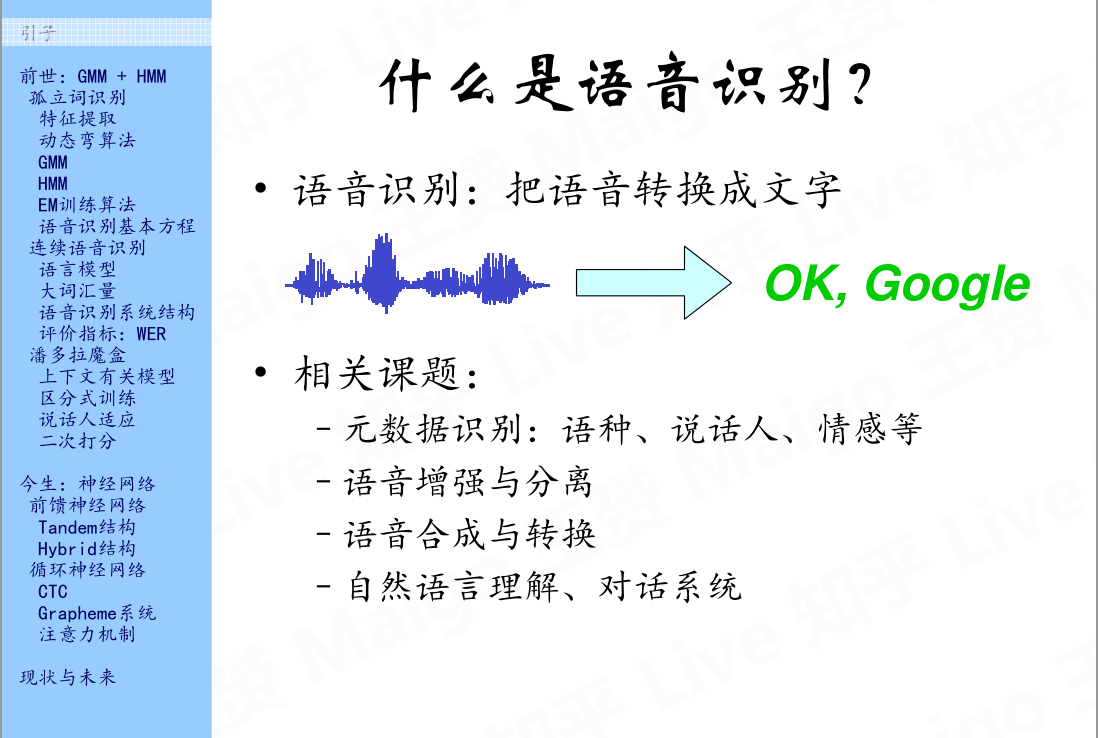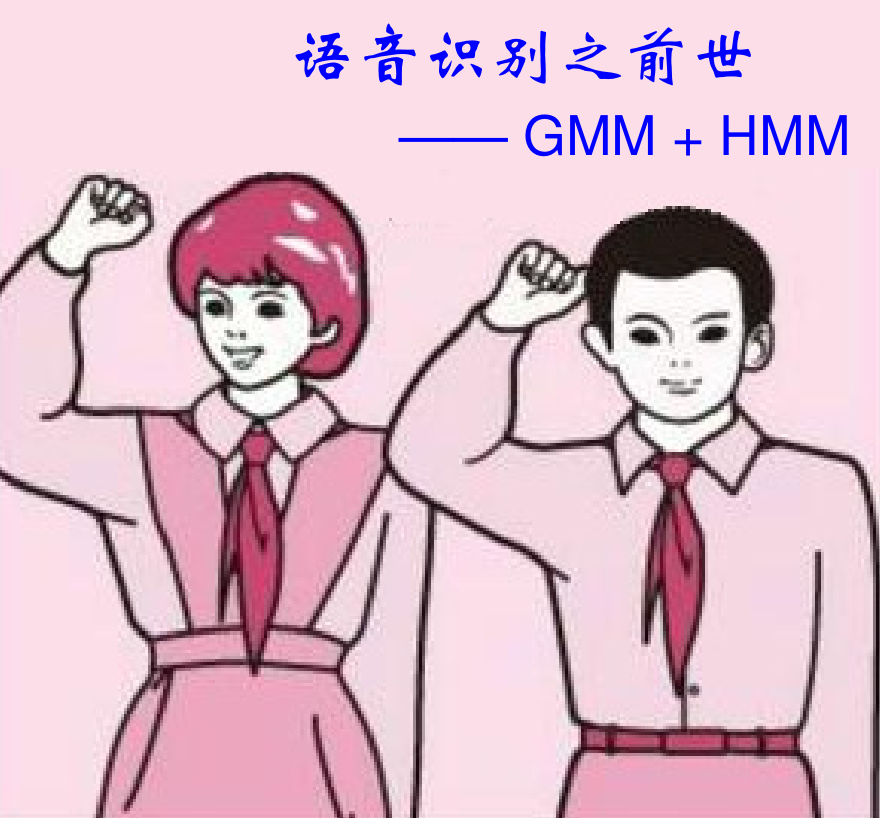# 2.孤立词识别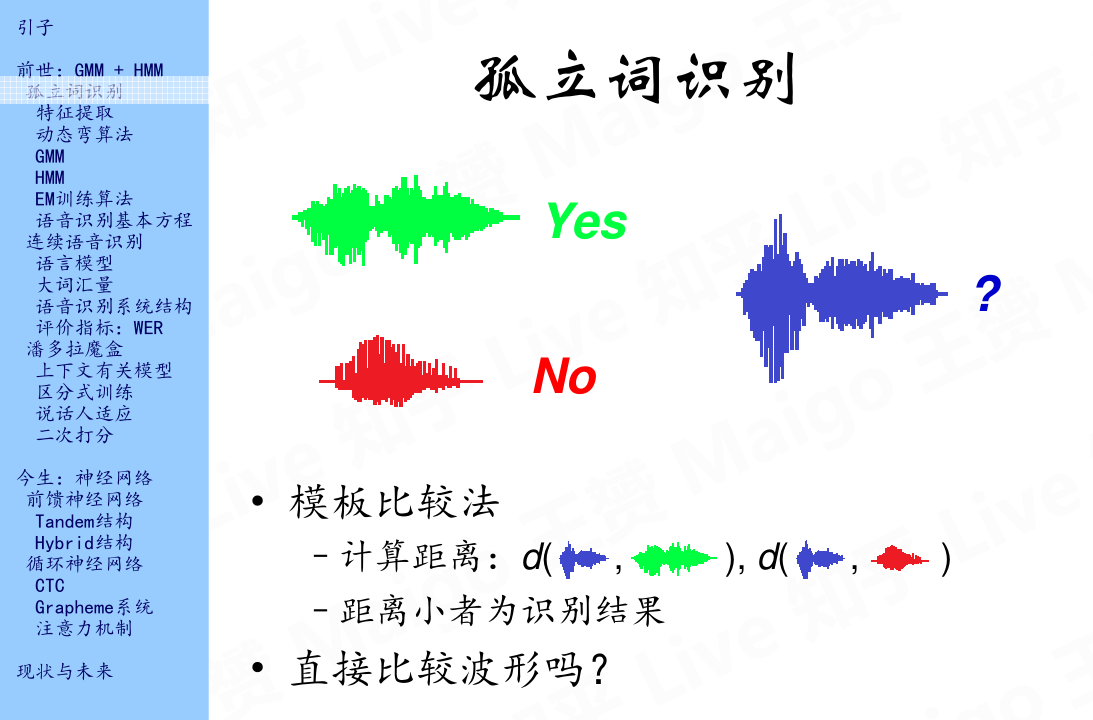## 2.1 特征提取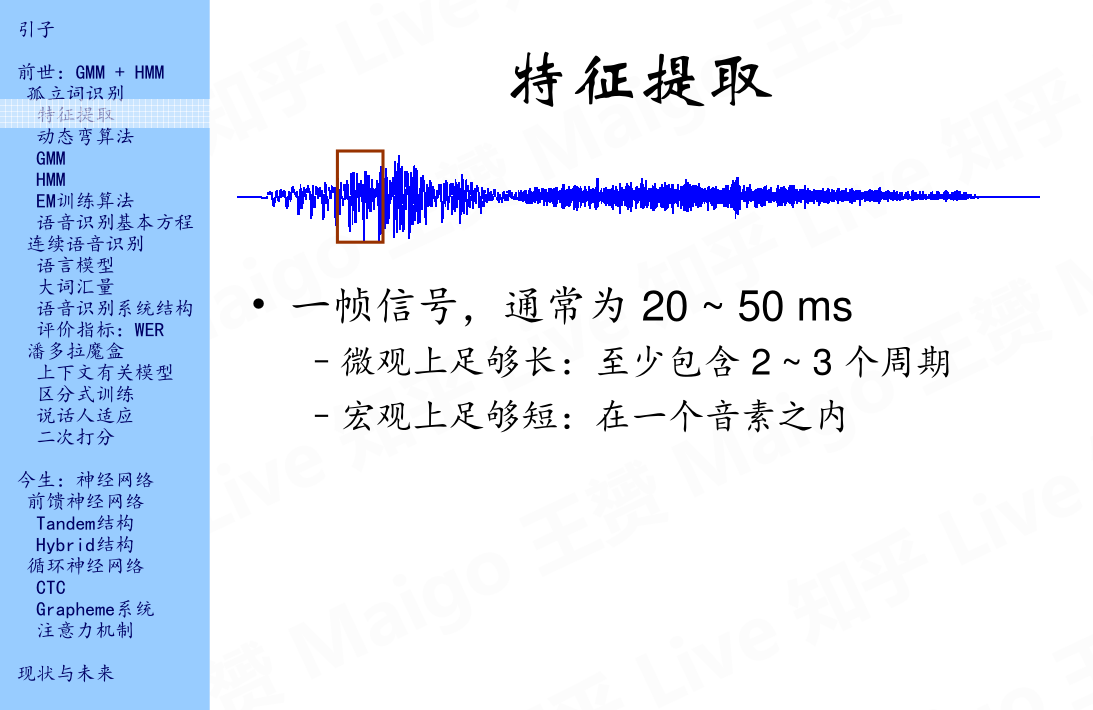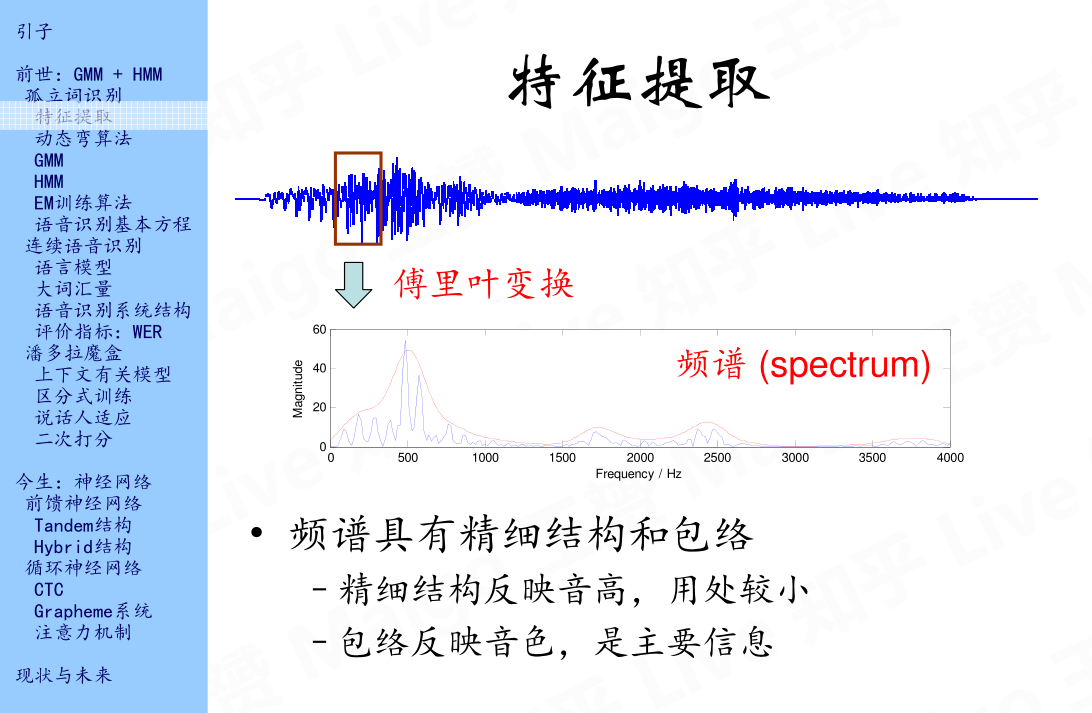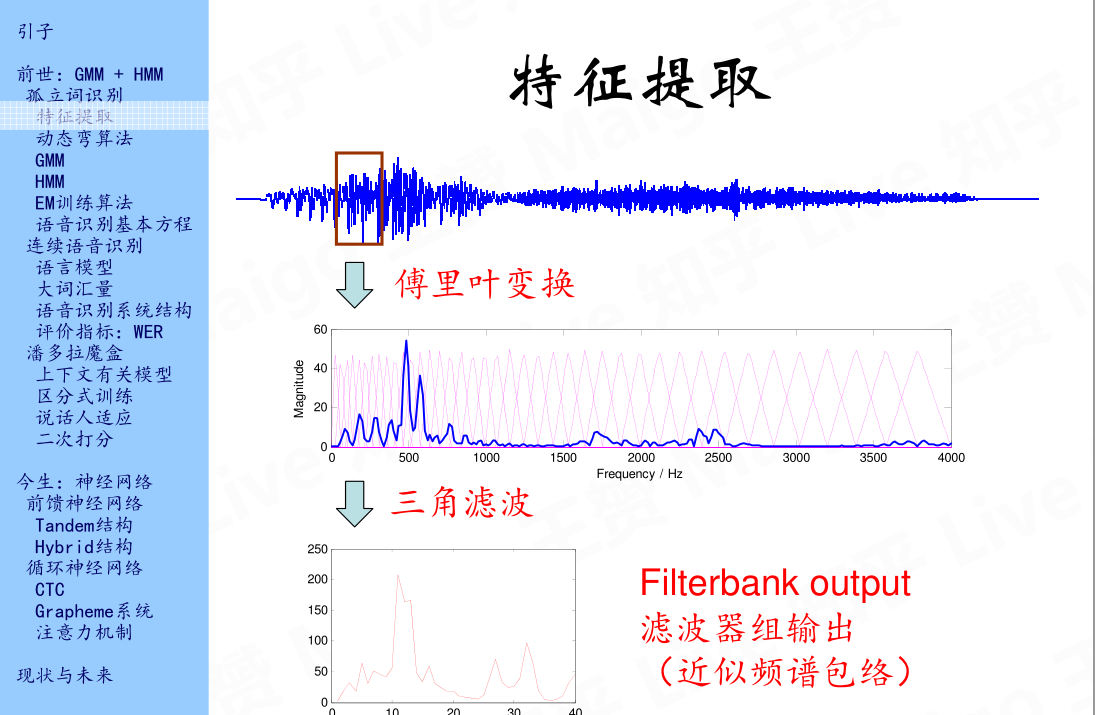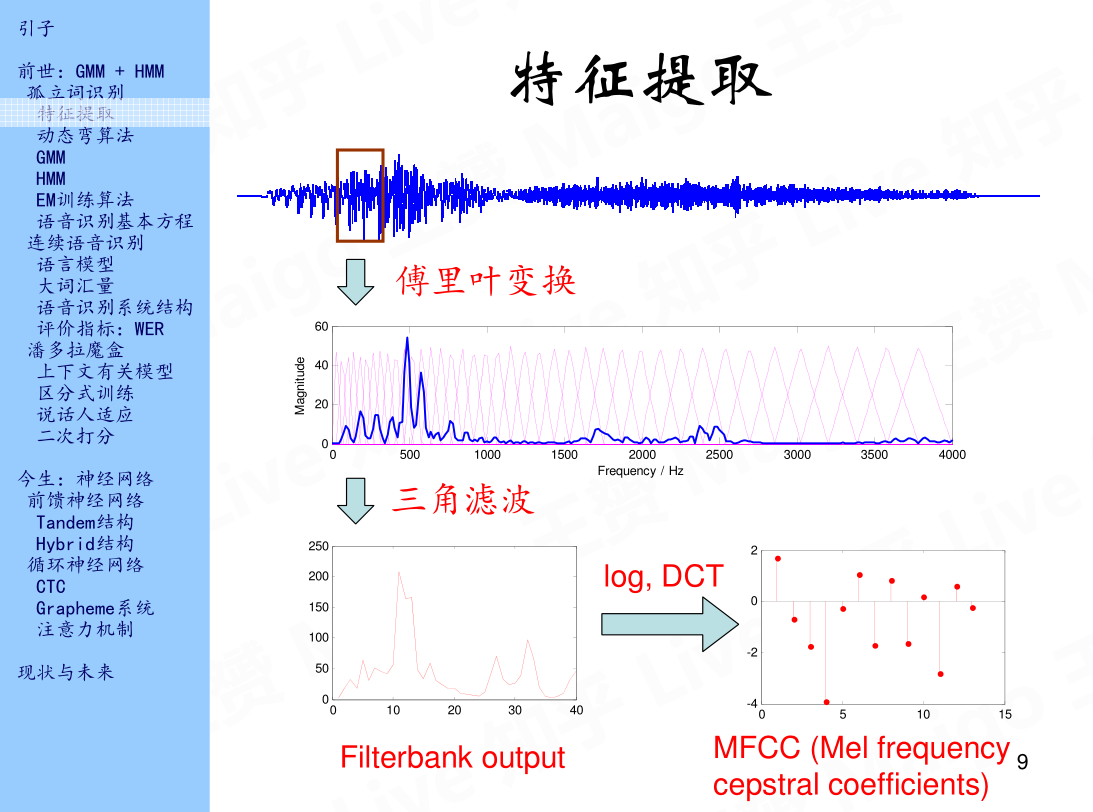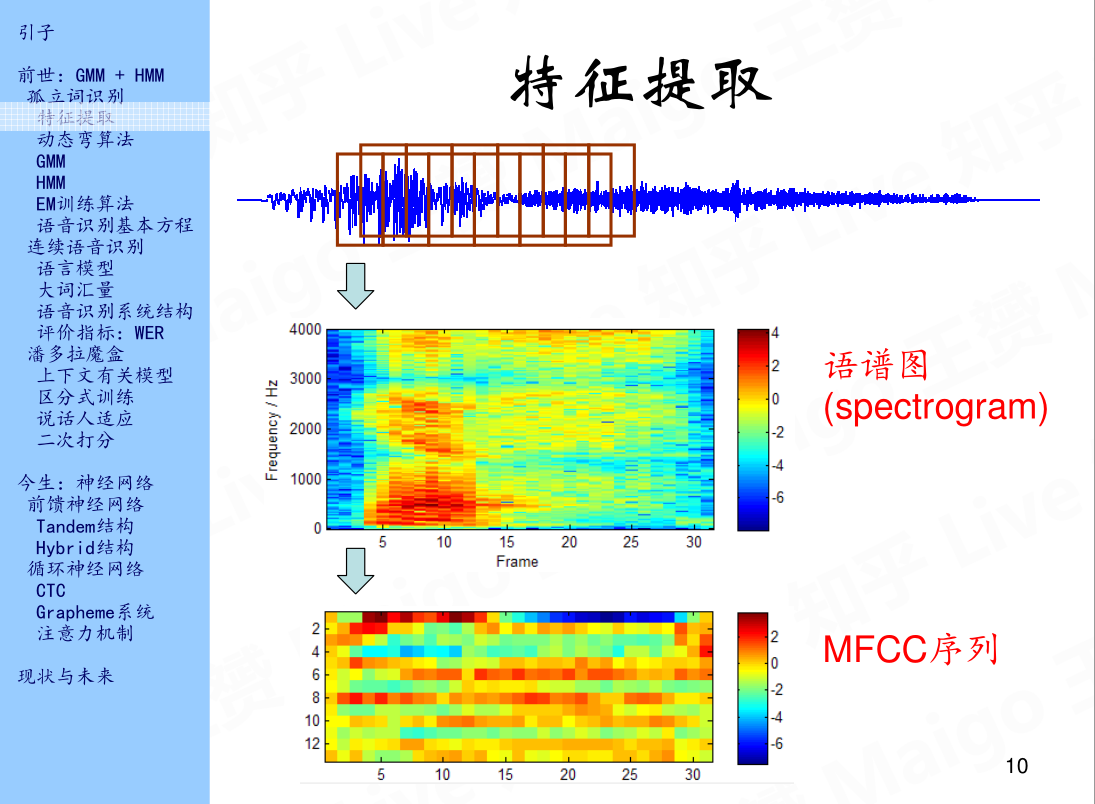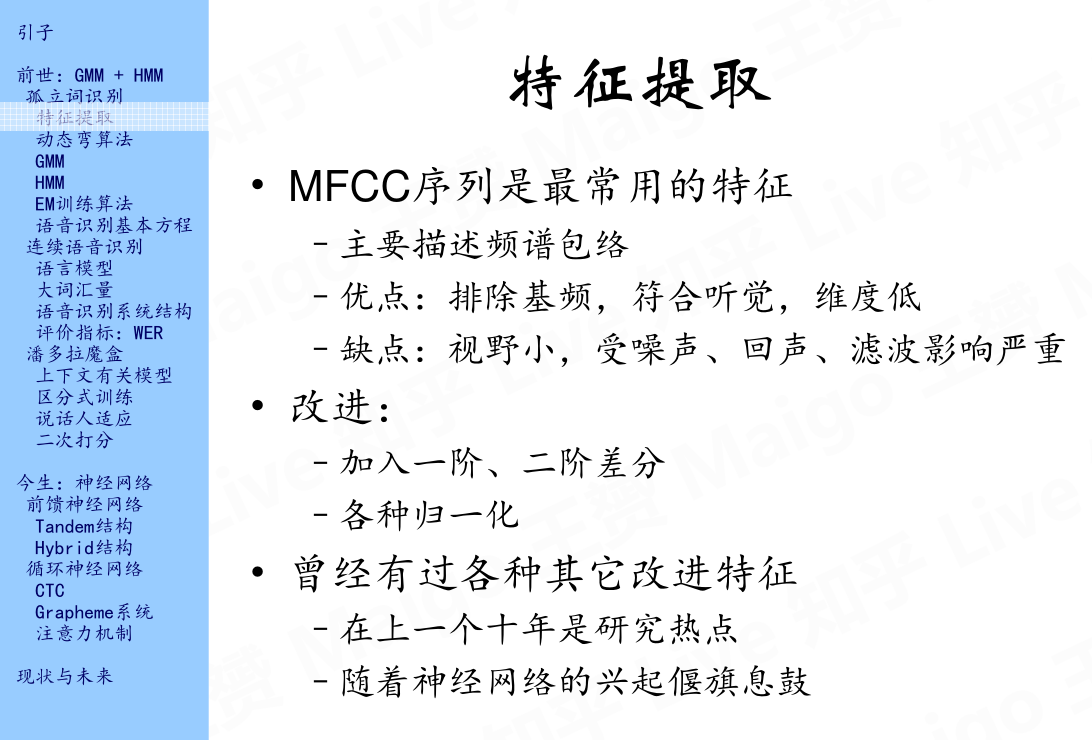## 2.2 动态弯算法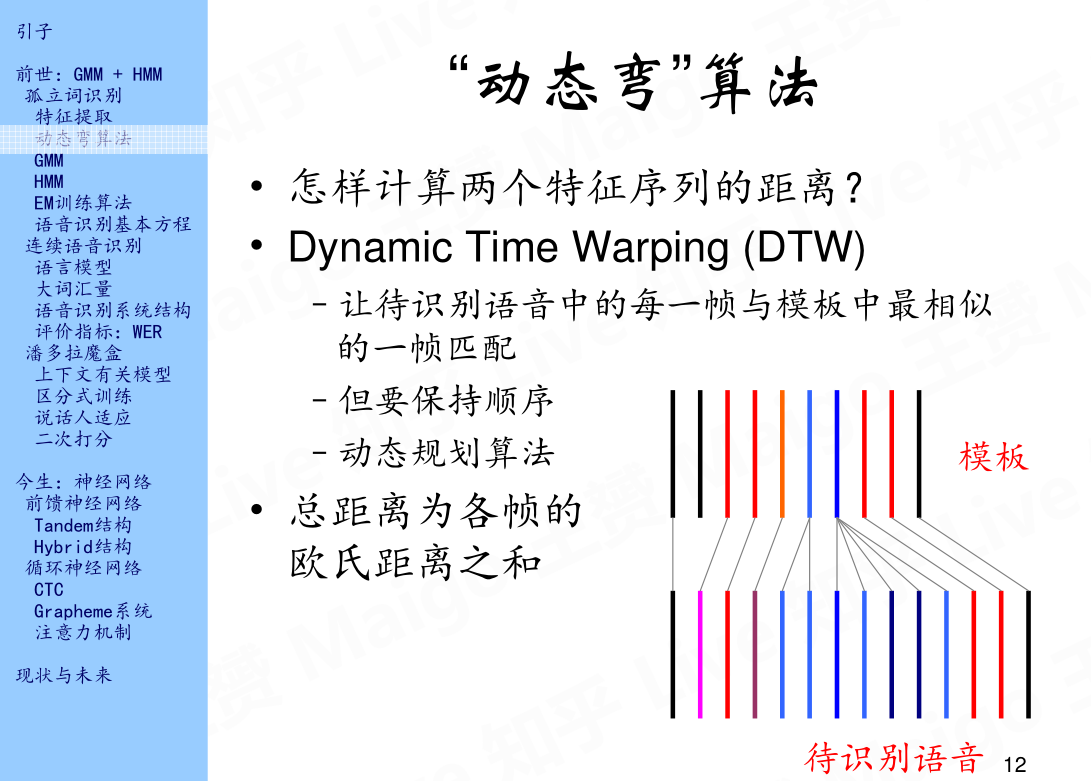## 2.3 GMM(Gaussian mixture model)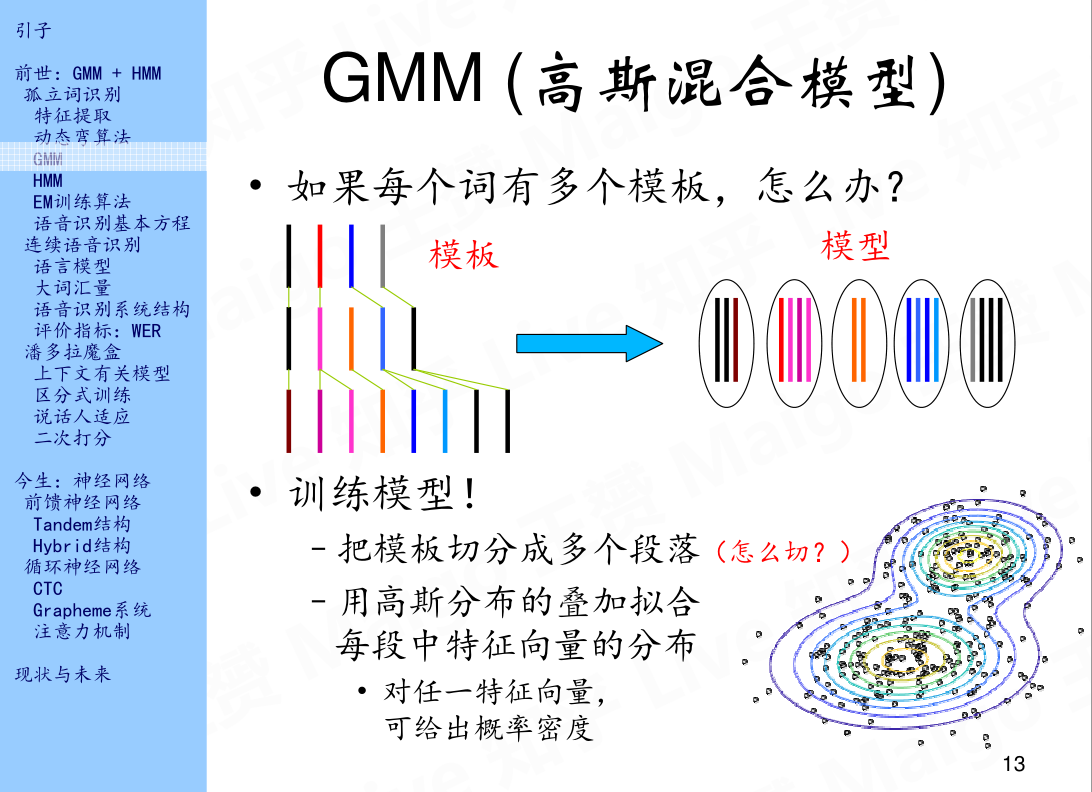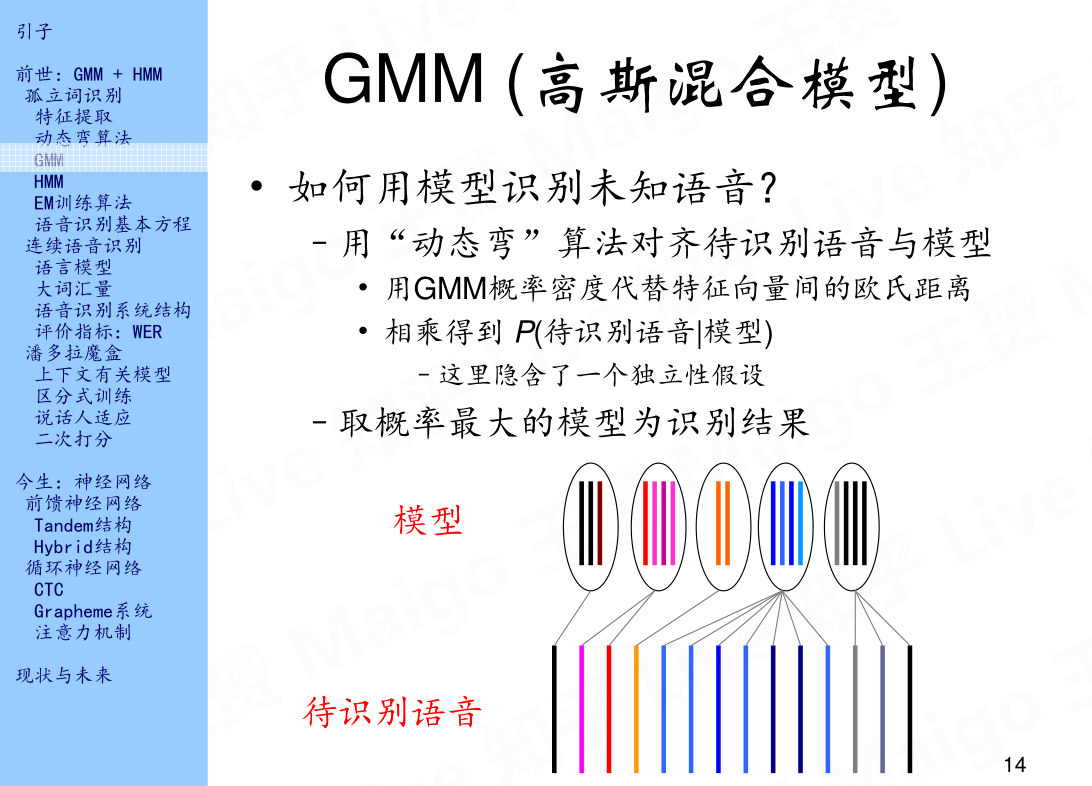## 2.4 HMM(Hidden markov model)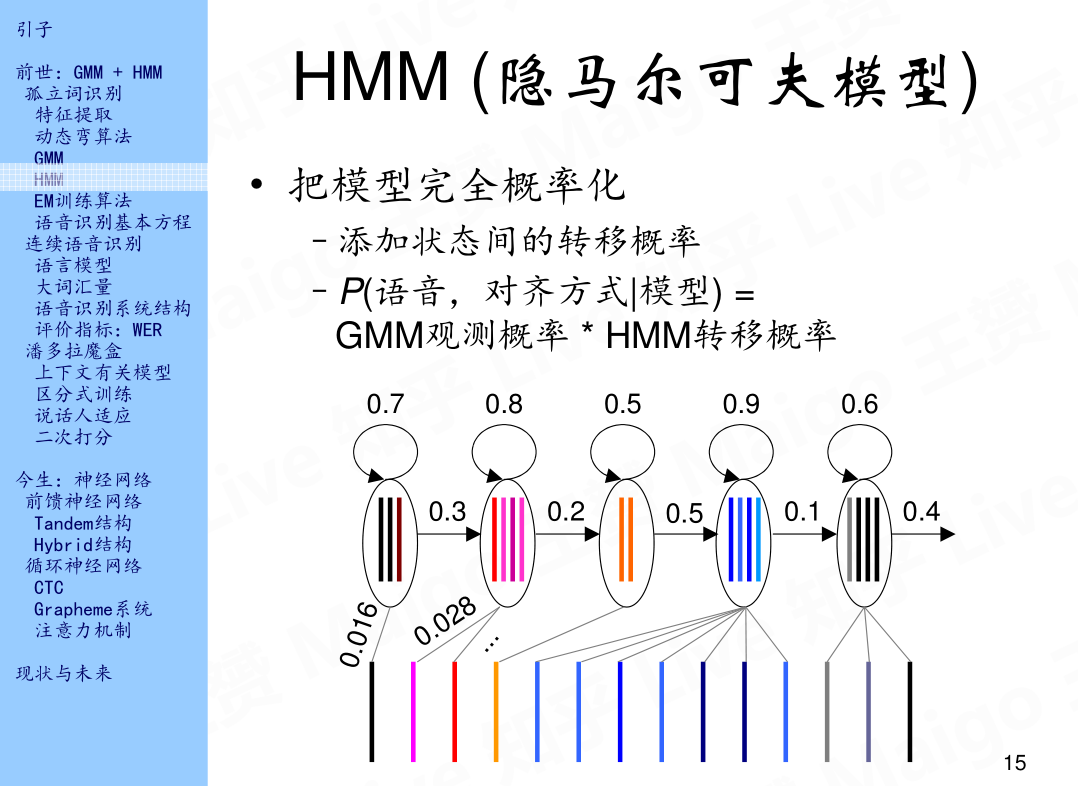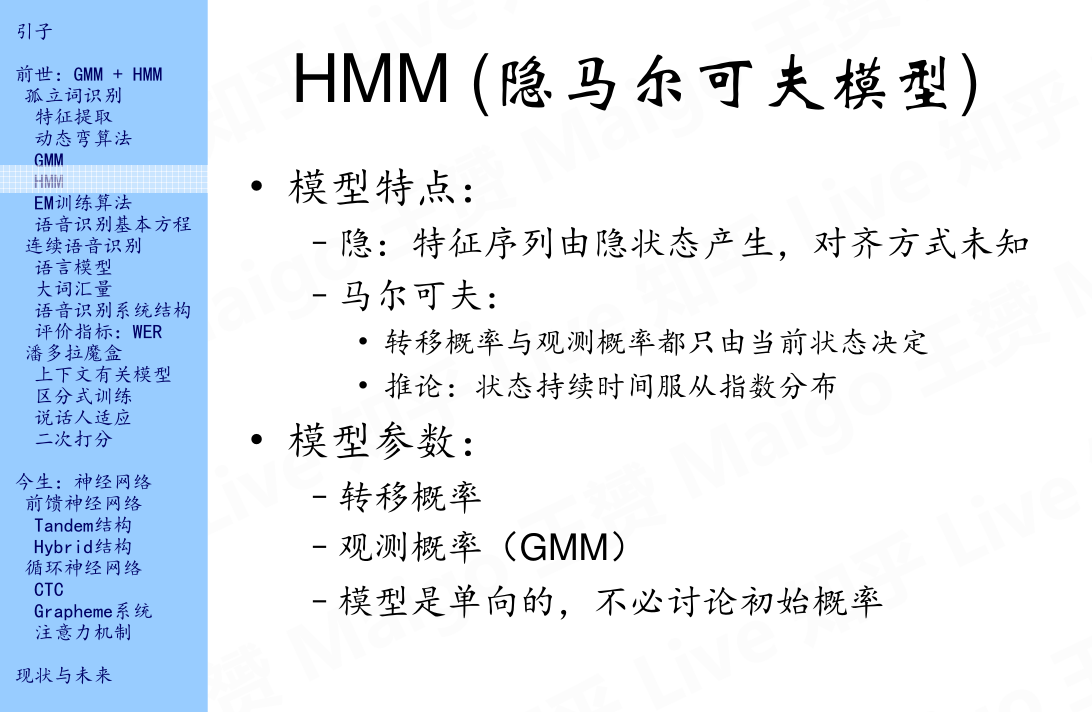HMM主要有两个特点，第一个就是特征序列由隐状态产生，隐状态也就是上面说到的五个状态，为什么称之为隐状态呢，是因为每帧特征向量和状态之间的对齐方式是未知的；另一个特点就是马尔可夫性，这里就不多说了。有关GMM，HMM，包括下面将要说的EM的相关知识，我推荐大家可以看一下悉尼科技大学的徐亦达老师在优酷的一个自频道，里面详细的讲解了概率统计的一些知识，并且每一个概念都有详细的公式推导，值得一看，教学视频见参考文献3。说完模型的特点，接下来说一下模型的参数，实际上HMM是有三个参数的：转移概率，观测概率以及初始概率。但是上面示例中的HMM是单向的，所以初始状态默认为第一个状态，所以，模型的参数就变成了两个：转移概率和观测概率。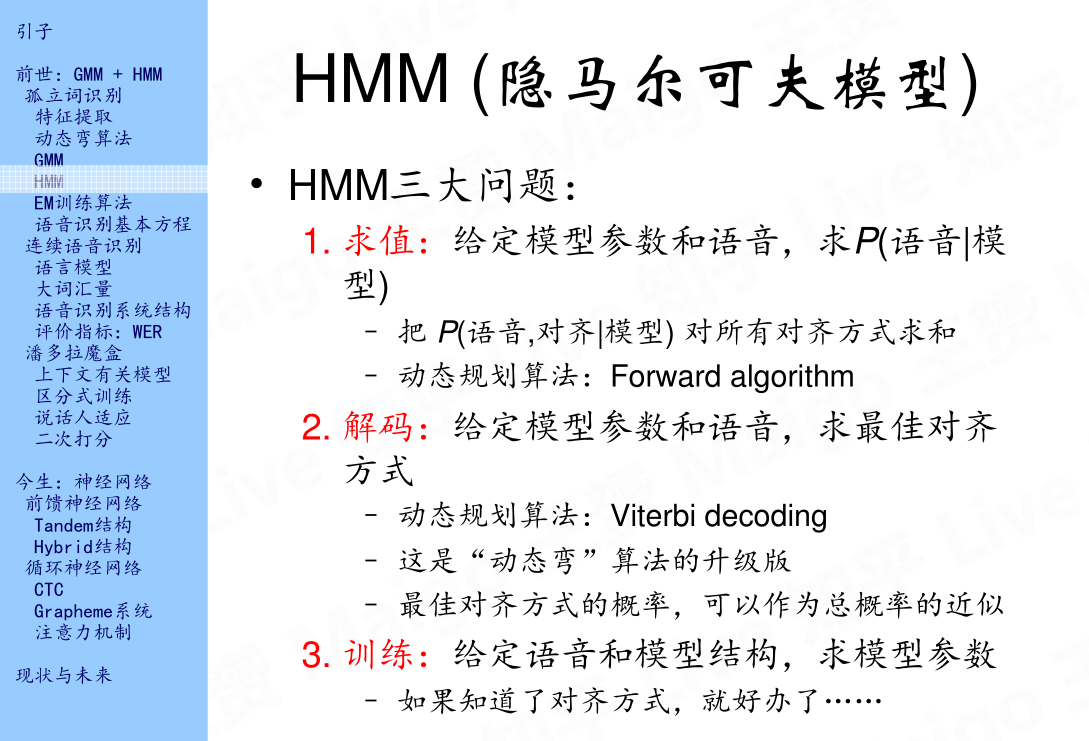## 2.5 EM训练算法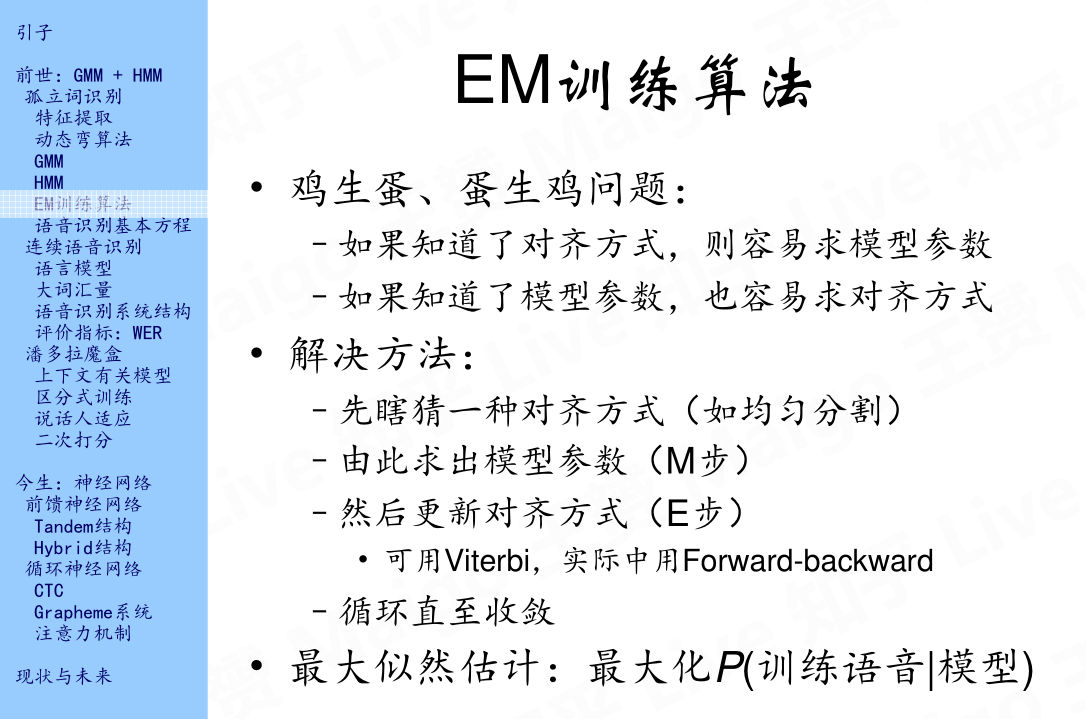## 2.6 语音识别基本方程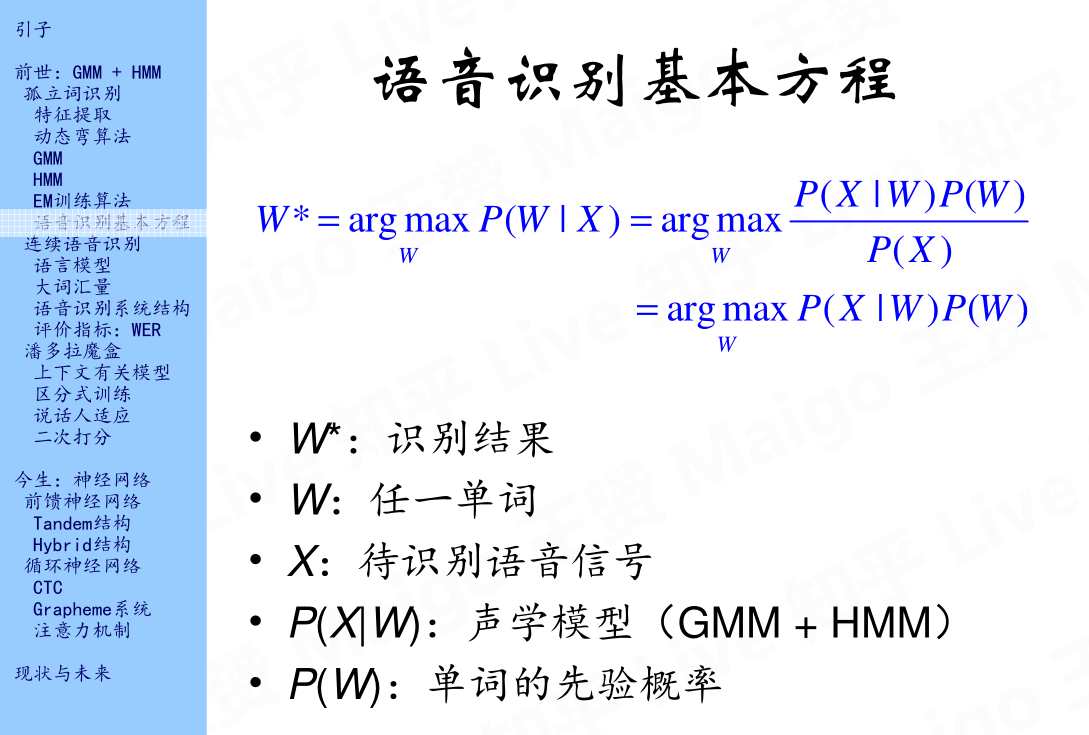# 3.连续语音识别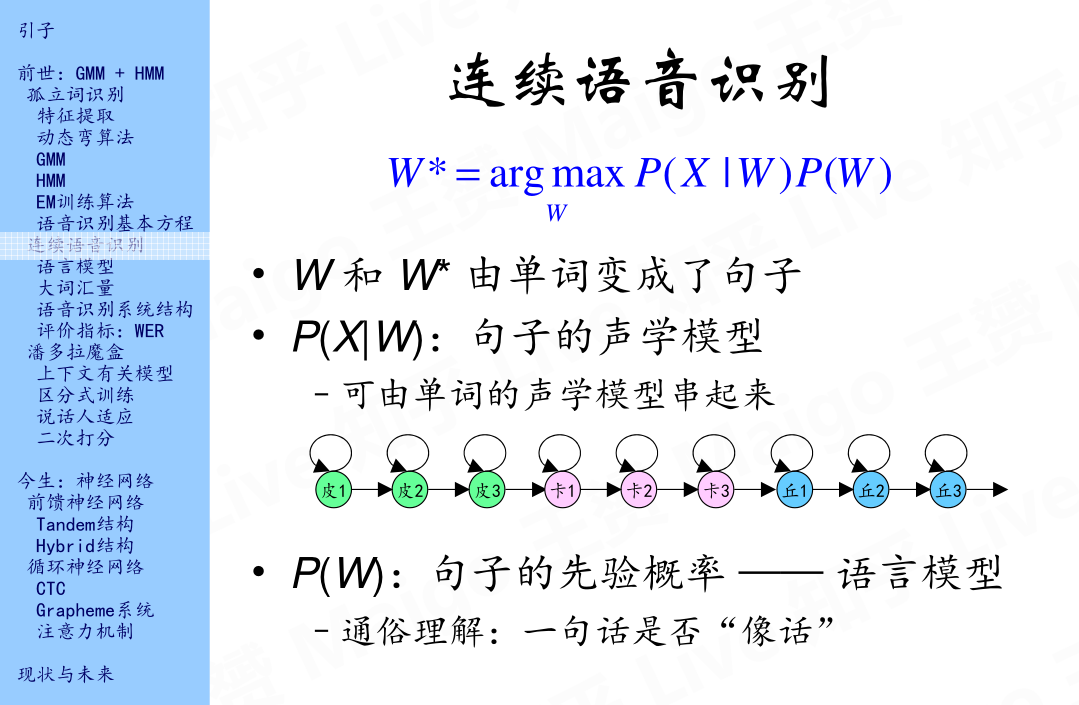## 3.1 语言模型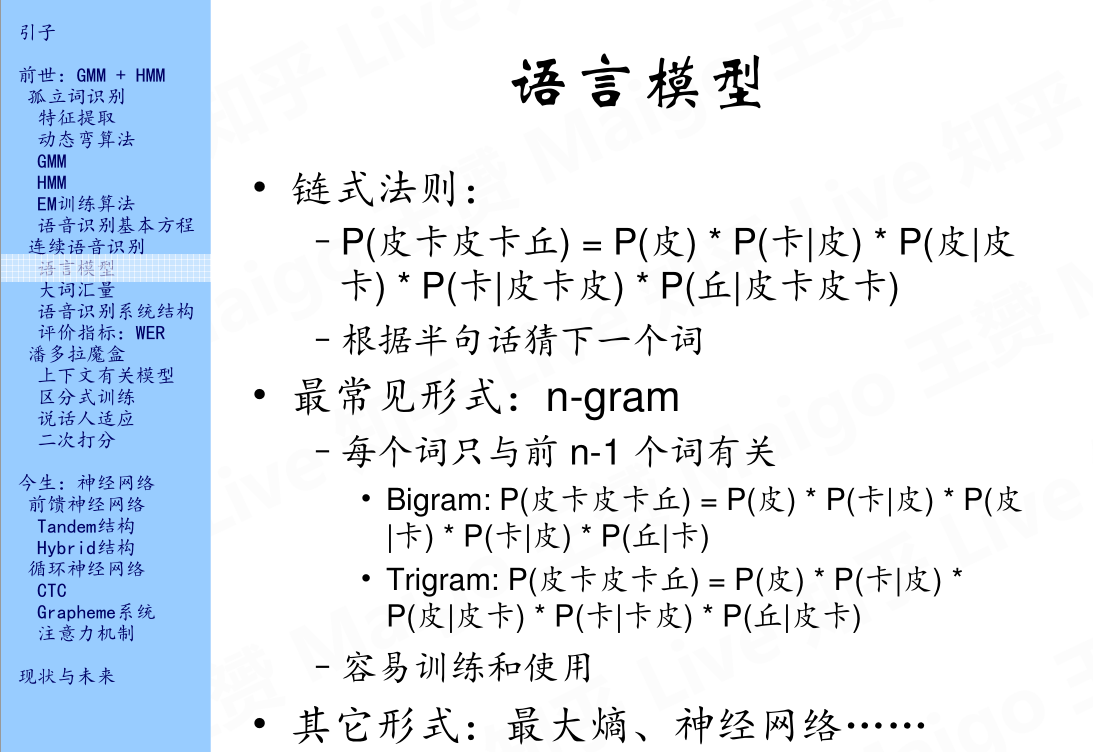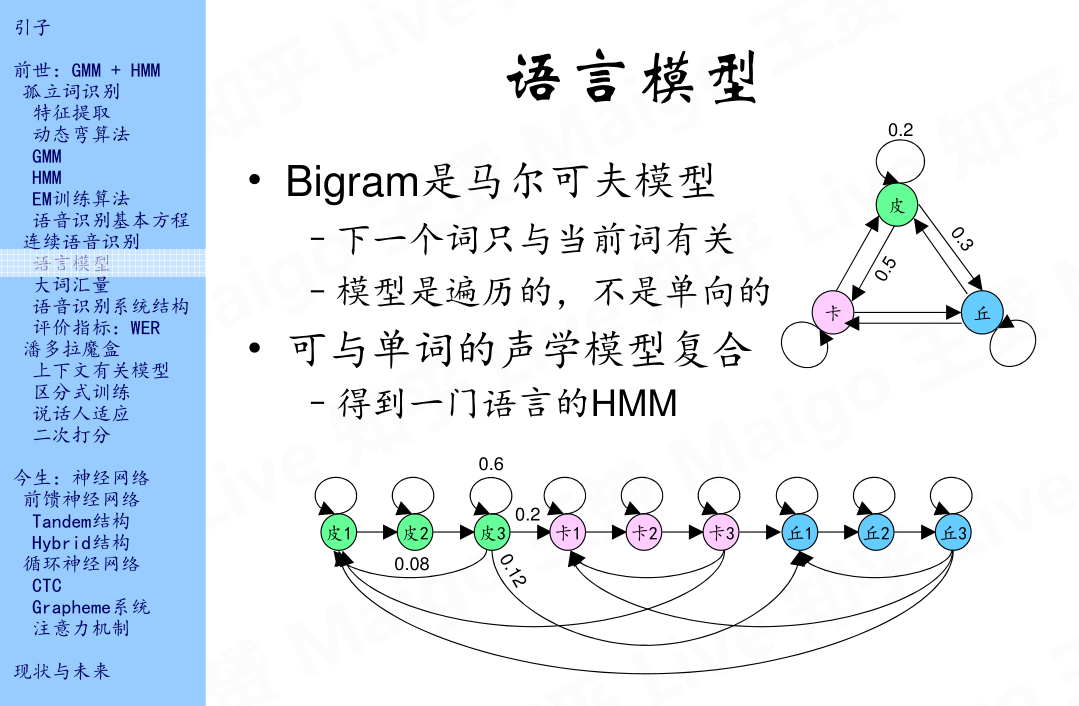## 3.2 大词汇量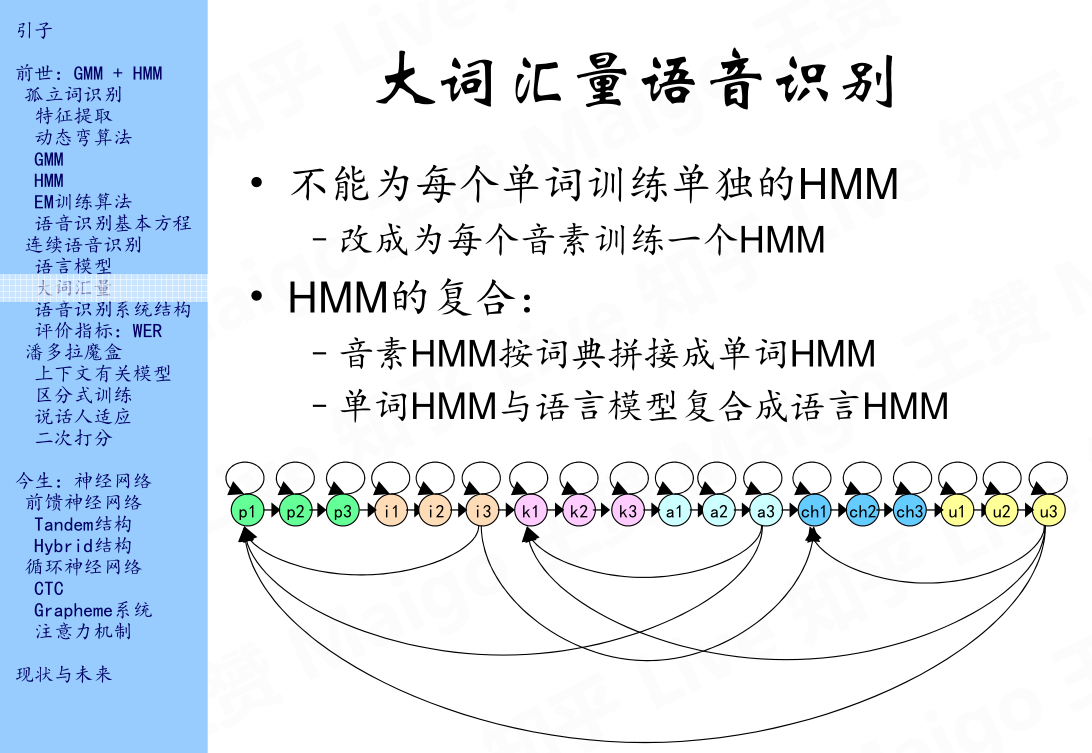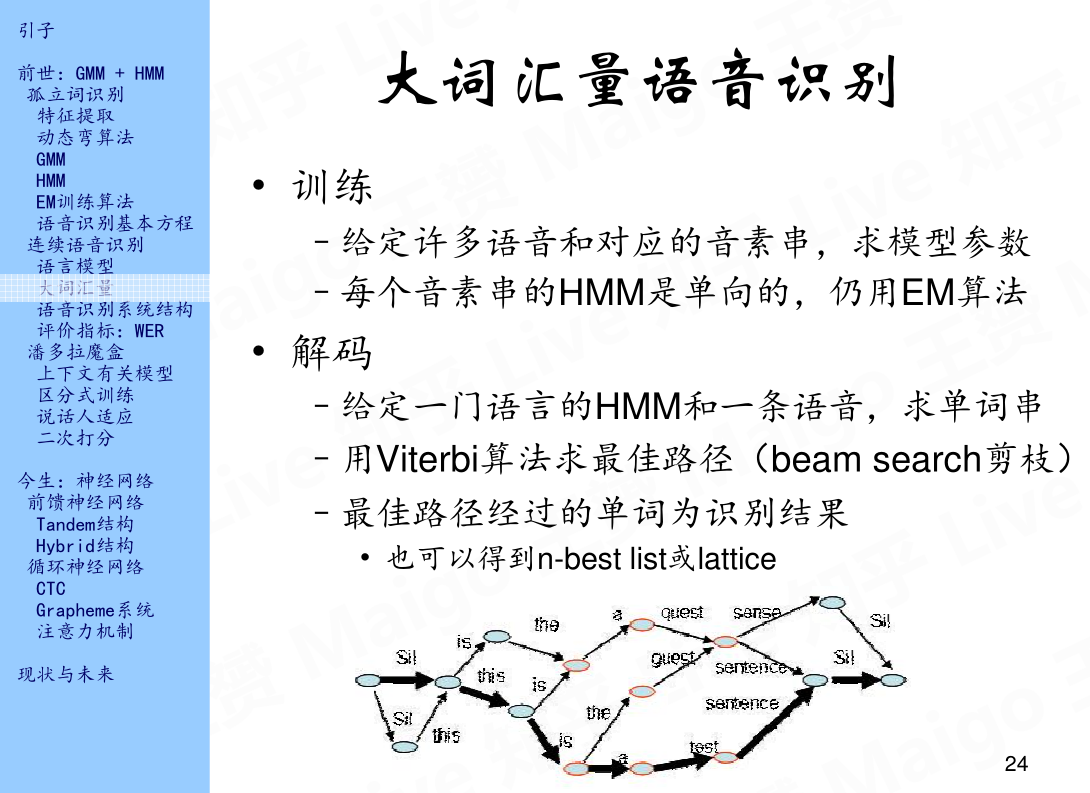## 3.3 语音识别系统结构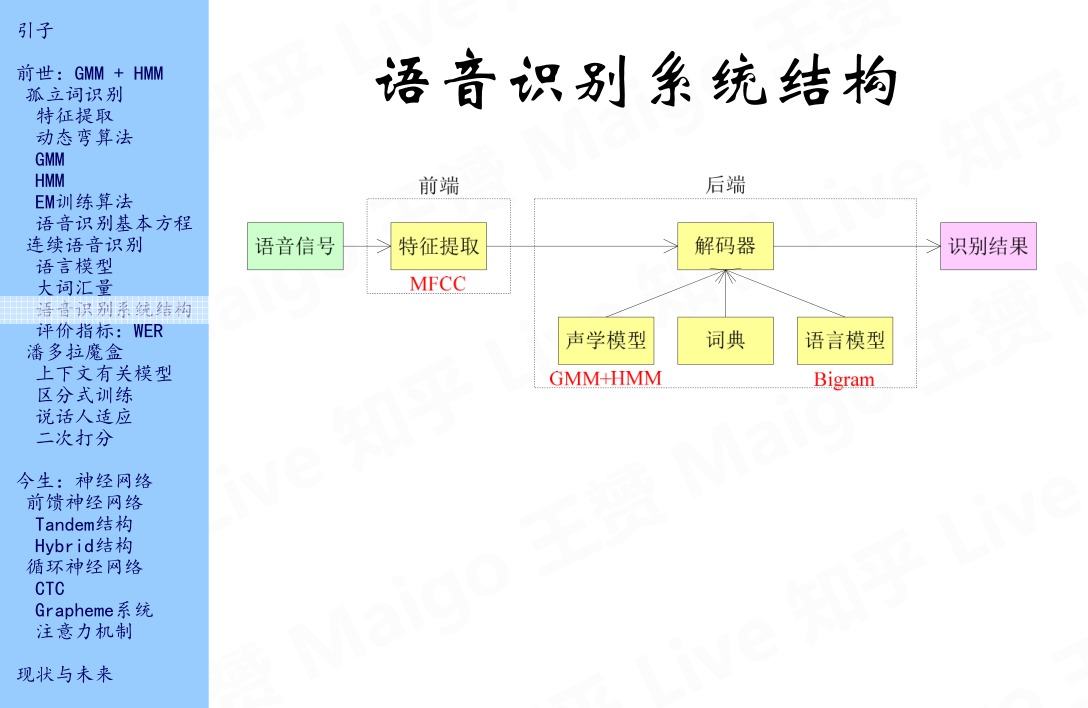## 3.4 评价指标：WER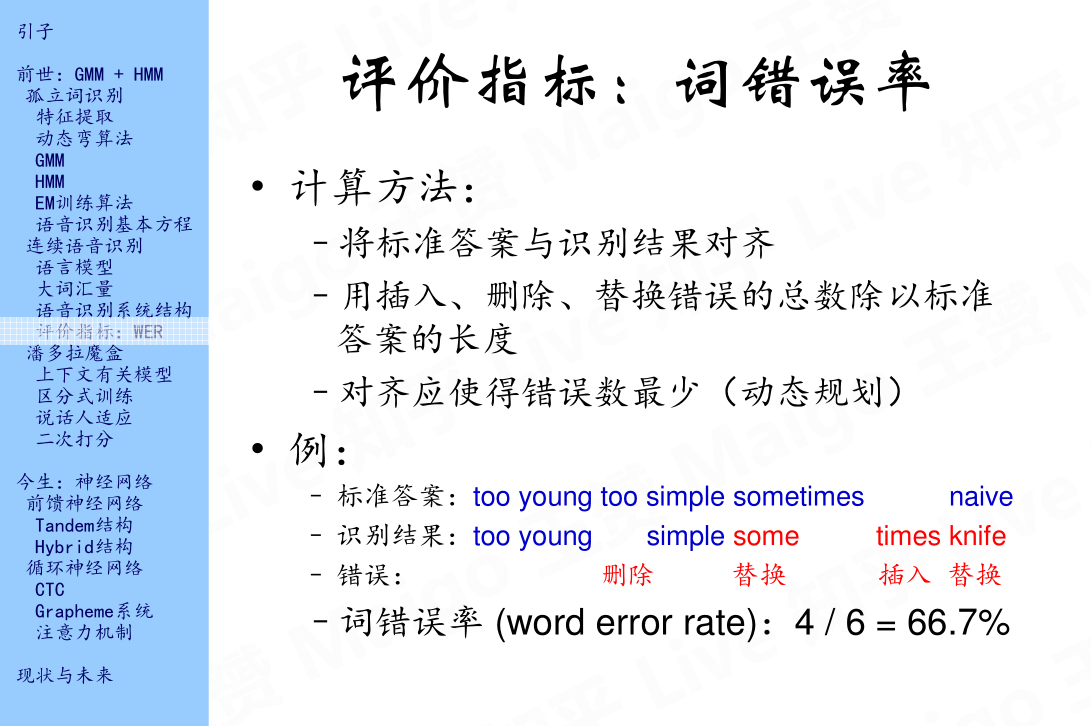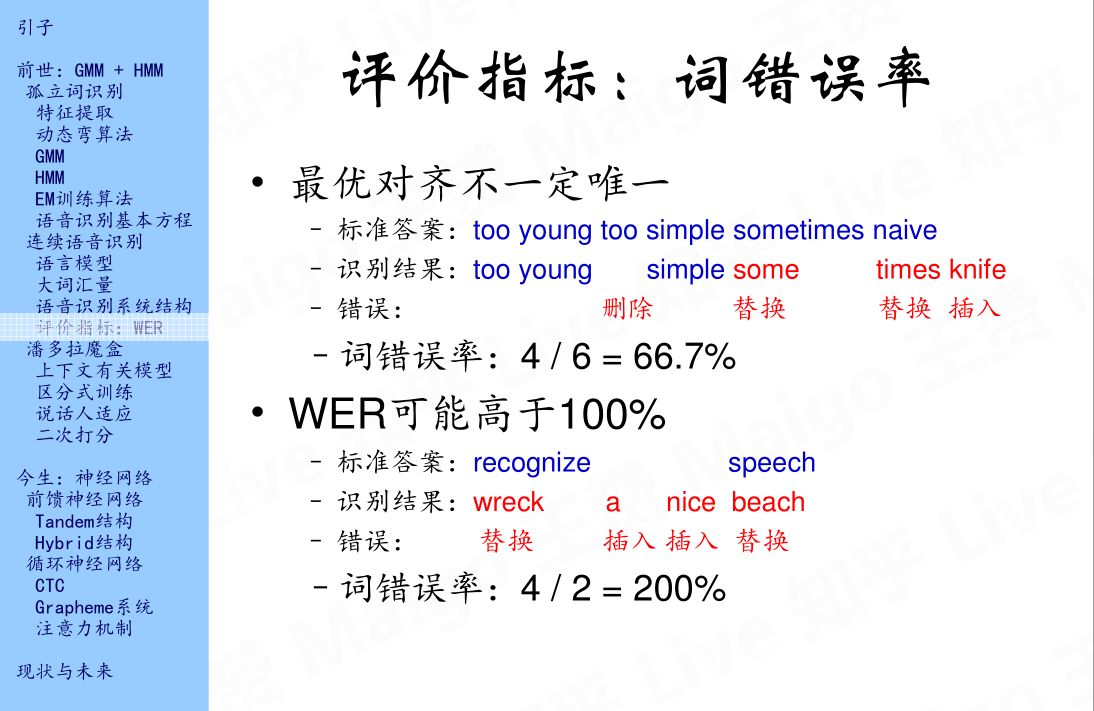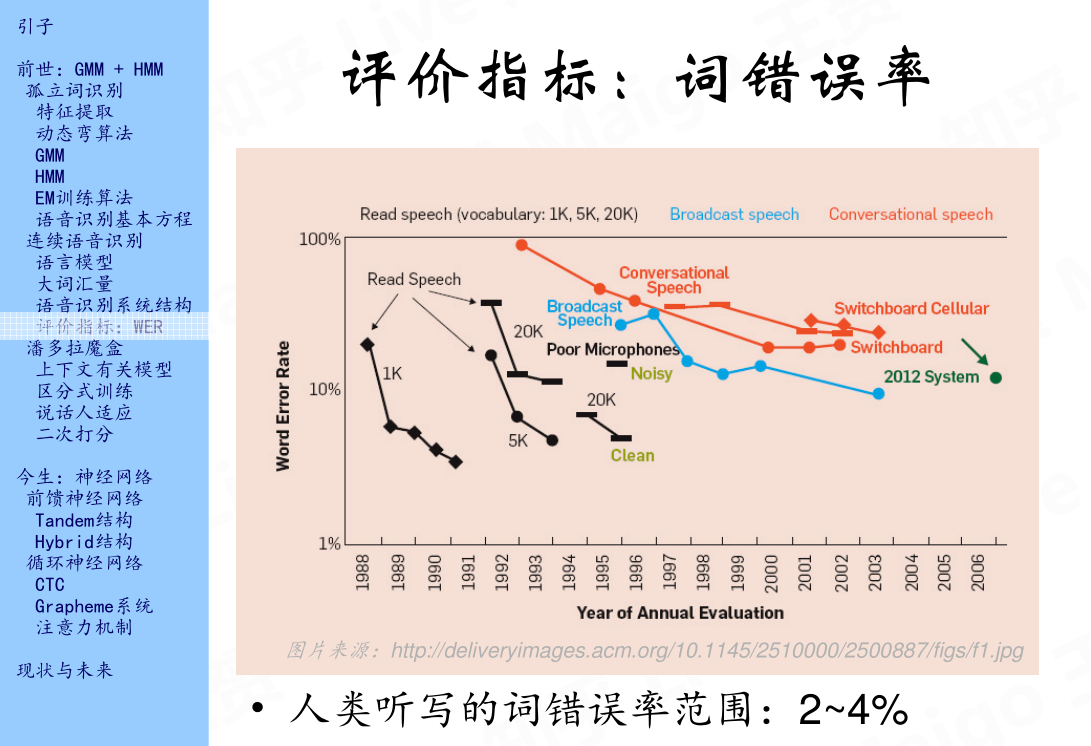# 4. 潘多拉魔盒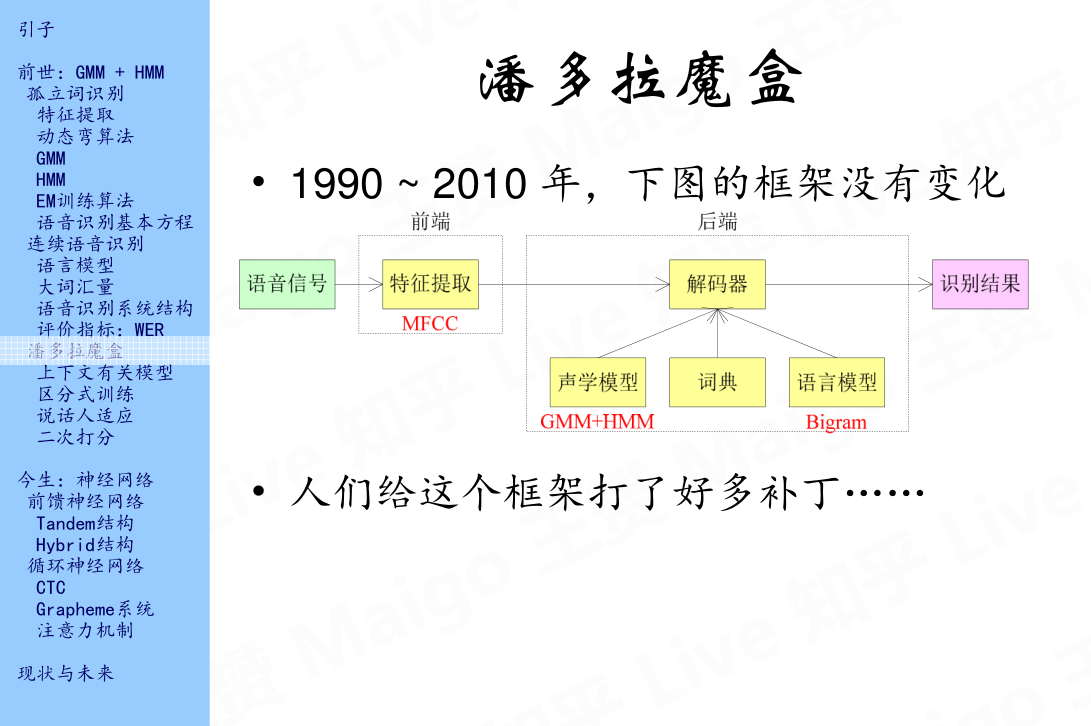## 4.1 上下文有关模型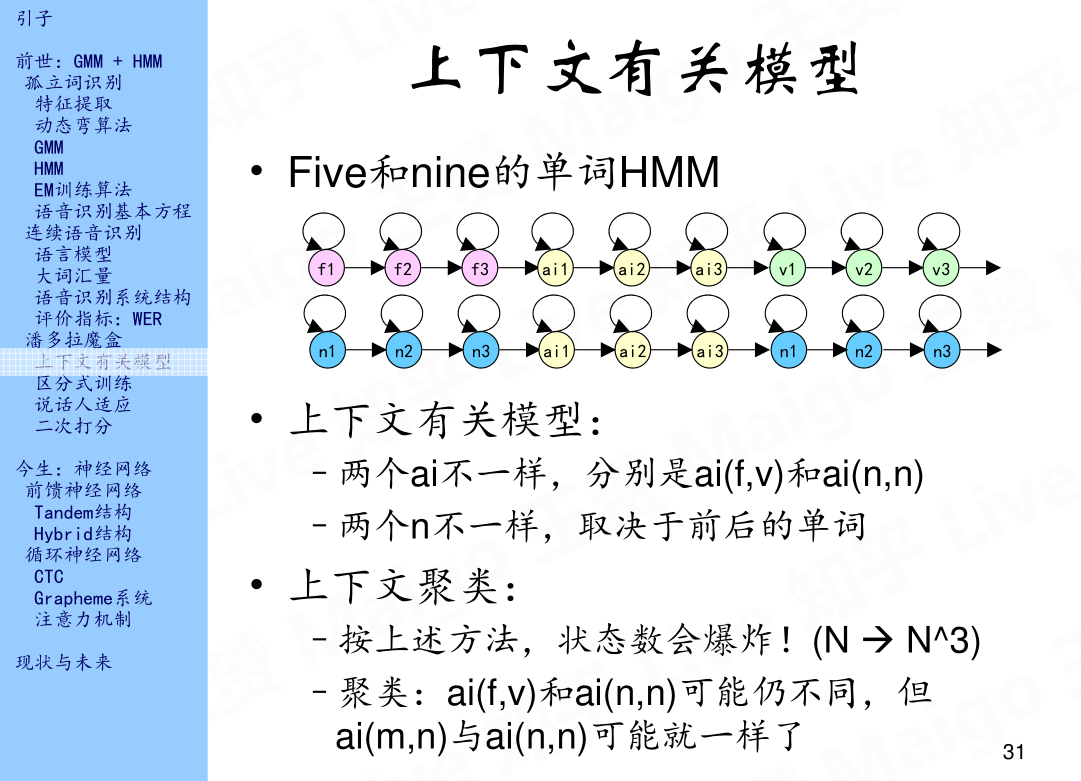## 4.2 区分式训练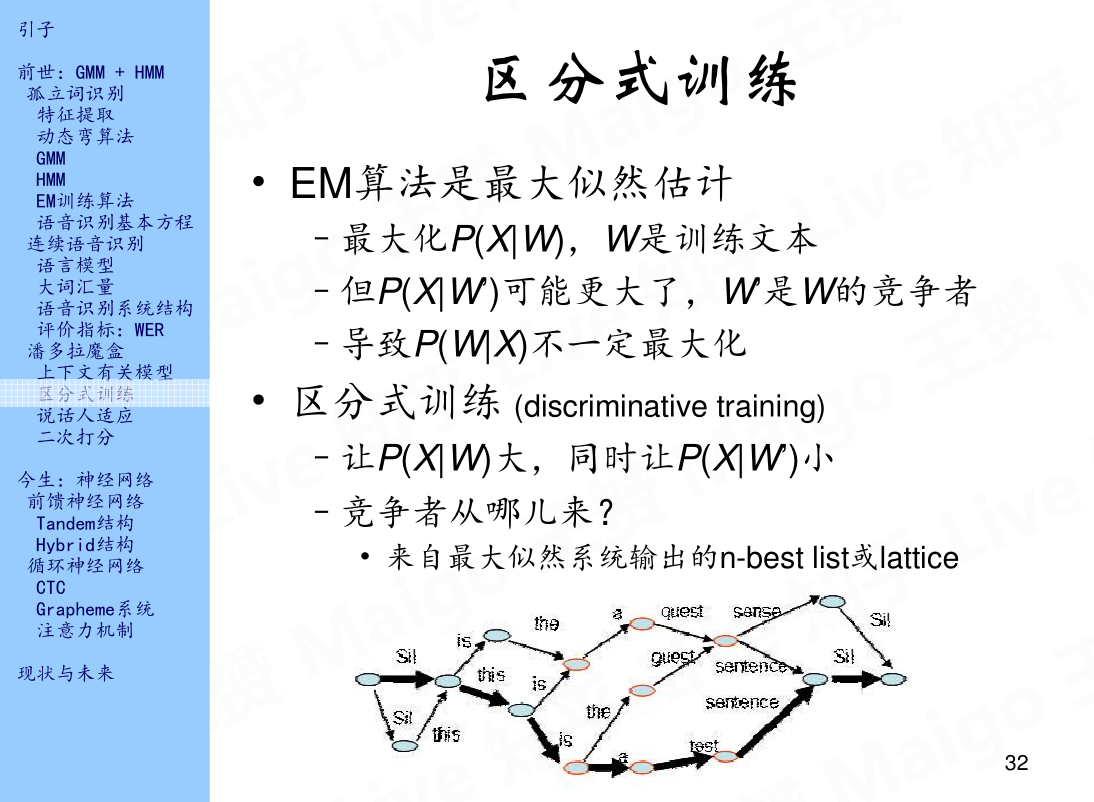## 4.3 说话人适应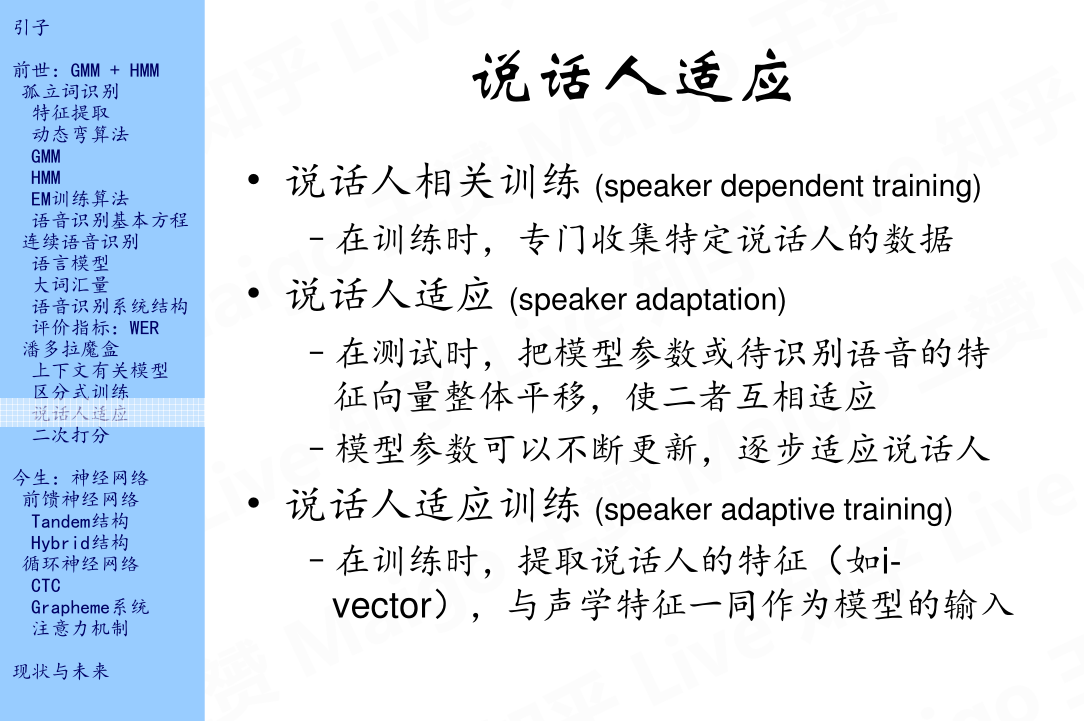## 4.4 二次打分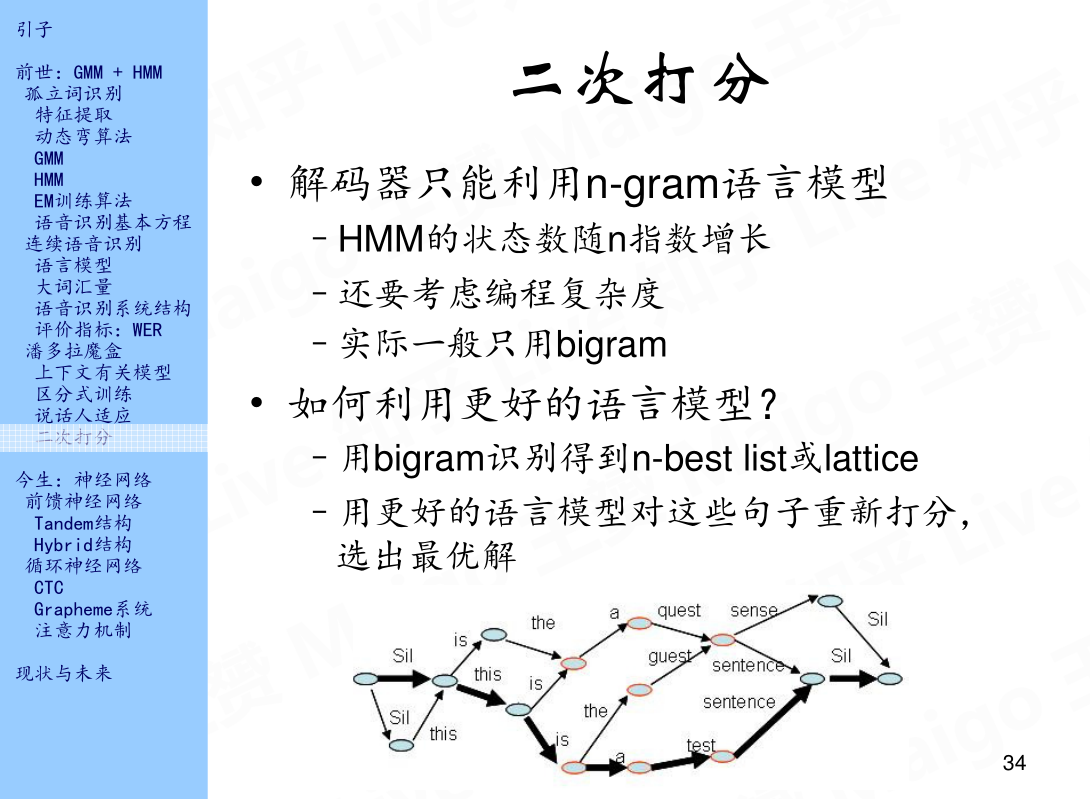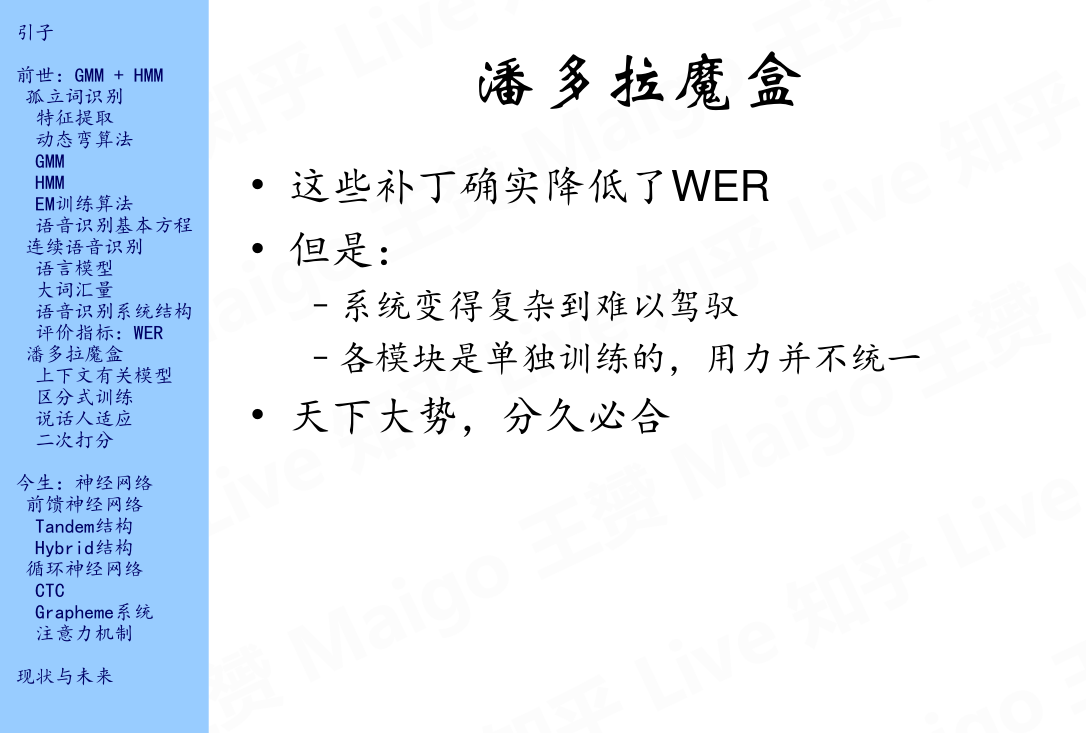# 参考文献

1、语音识别技术的前世今生：https://www.bilibili.com/video/av19158521/

2、傅里叶变换：https://zhuanlan.zhihu.com/p/19763358

3、徐亦达自频道：http://i.youku.com/i/UMzIzNDgxNTg5Ng==?spm=a2hzp.8253869.0.0

2017-04-04 17:29:52 u013131595 阅读数 565
• ###### C++语音识别开篇

本篇mark老师将教大家使用第三方库的调用来简单的实现语音识别。随着机器学习和人工智能的热闹，国内语音行业也可谓是百花齐放。 语音识别一个伟大的时代已在我们身边悄悄走来。

6113 人正在学习 去看看 杨波

# 1.调用关系图

def read_datasets(conf_path, sets, numcep, numcontext,
data_dir, dataset_config = _get_data_set_dict(conf_path, sets)

path = os.path.join(data_dir, config['dir_pattern'])
return DataSet.from_directory(path,
batch_size=config['batch_size'],
numcep=numcep,
numcontext=numcontext,
start_idx=config['start_idx'],
limit=config['limit'],
sort=config['sort']
)
if name in sets else None
for name in ('train', 'dev', 'test')}
#sets传入的值为['train', 'dev', 'test']
return DataSets(**datasets)

_get_data_set_dict函数代码如下图所示：

def _get_data_set_dict(conf_path, sets):
parser = ConfigParser(os.environ)
data_dict = {}

if 'train' in sets:
d = {}
data_dict['train'] = d
logging.debug('Training configuration: %s', str(d))

if 'dev' in sets:
d = {}
data_dict['dev'] = d
logging.debug('Dev configuration: %s', str(d))

if 'test' in sets:
d = {}
data_dict['test'] = d
logging.debug('Test configuration: %s', str(d))

return data_dir, data_dict# 3.from_directory函数

DataSet类中起主要作用的函数为from_directory函数和next_batch函数。from_directory函数用来从data目录下构建之后需要用到的DataSet对象，具体代码如下：

    def from_directory(cls, dirpath, thread_count, batch_size, numcep, numcontext, start_idx=0, limit=0, sort=None):
if not os.path.exists(dirpath):
raise IOError("'%s' does not exist" % dirpath)
txt_files = txt_filenames(dirpath, start_idx=start_idx, limit=limit, sort=sort)
if len(txt_files) == 0:
raise RuntimeError('start_idx=%d and limit=%d arguments result in zero files' % (start_idx, limit))
return cls(txt_files, thread_count, batch_size, numcep, numcontext)

def txt_filenames(dataset_path, start_idx=0, limit=None, sort='alpha'):
# Obtain list of txt files
txt_files = glob(os.path.join(dataset_path, "*.txt"))
limit = limit or len(txt_files)

# Optional: sort files to improve padding performance
if sort not in SORTS:
raise ValueError('sort must be one of [%s]', SORTS)
reverse = False
key = None
if 'filesize' in sort:
key = os.path.getsize
if sort == 'filesize_high_low':
reverse = True
elif sort == 'random':
key = lambda *args: random()
txt_files = sorted(txt_files, key=key, reverse=reverse)

return txt_files[start_idx:limit + start_idx]

# 4.next_batch函数

    def next_batch(self, batch_size=None):
if batch_size is None:
batch_size = self._batch_size

end_idx = min(len(self._txt_files), self._start_idx + batch_size)
idx_list = range(self._start_idx, end_idx)
txt_files = [self._txt_files[i] for i in idx_list]
wav_files = [x.replace('.txt', '.wav') for x in txt_files]
(source, _, target, _) = get_audio_and_transcript(txt_files,
wav_files,
self._numcep,
self._numcontext)

self._start_idx += batch_size
# Verify that the start_idx is not larger than total available sample size
if self._start_idx >= self.size:
self._start_idx = 0

# Pad input to max_time_step of this batch
sparse_labels = sparse_tuple_from(target)
return source, source_lengths, sparse_labels

2019-05-16 10:19:56 qq_35290785 阅读数 195
• ###### C++语音识别开篇

本篇mark老师将教大家使用第三方库的调用来简单的实现语音识别。随着机器学习和人工智能的热闹，国内语音行业也可谓是百花齐放。 语音识别一个伟大的时代已在我们身边悄悄走来。

6113 人正在学习 去看看 杨波

a. 步骤

b. 系统的设计

c . QA模型流程图

d. 体系结构和各个部分细分，

1.波形合成法

2.参数合成法

3.规则合成法

# 系统方案总述

## a. 步骤

1. 第一步，语音识别步骤，首先录入用户语言并进行识别，
2. 第二步 ，问答系统步骤，对识别出来的文本句子放入的问答系统中，提取答案，另外涉及到数据库的构建QA对的生成，模糊匹配问题，以及实时检索问题。
3. 第三步，语音合成步骤，将生成的答案，由文本转为语言形式展示给用户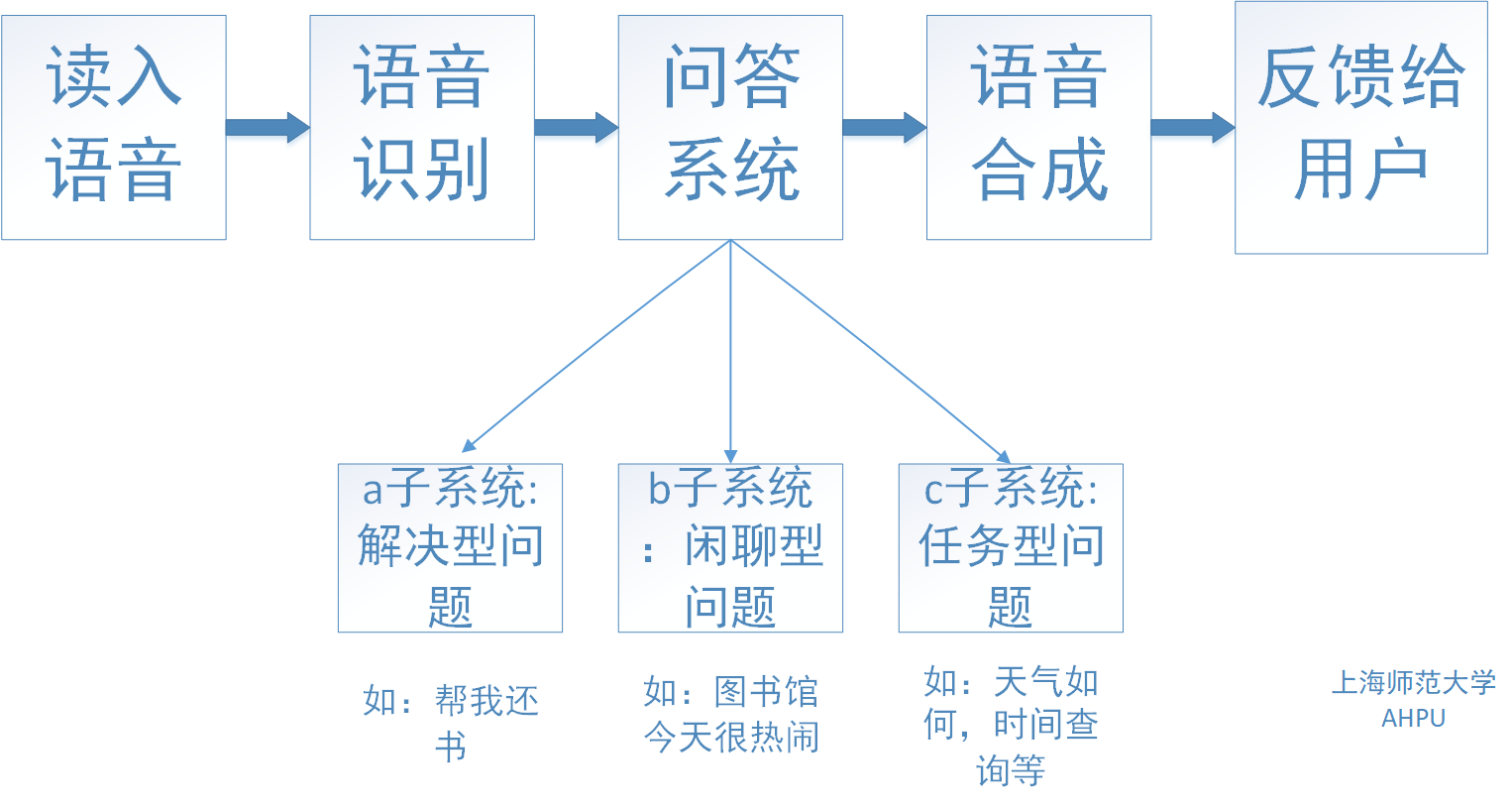## b. 系统的设计

1. A）：任务型机器人主要用于完成用户的某些特定任务，比如：查天气咨询，查时间。
2. B）：闲聊机器人主要用于深入的和用户进行无目的交流；
3. C）：解决型机器人（客服机器人）用于解决用户的问题，比如：查询书籍作者，剩余可借图书个数

## c . QA模型流程图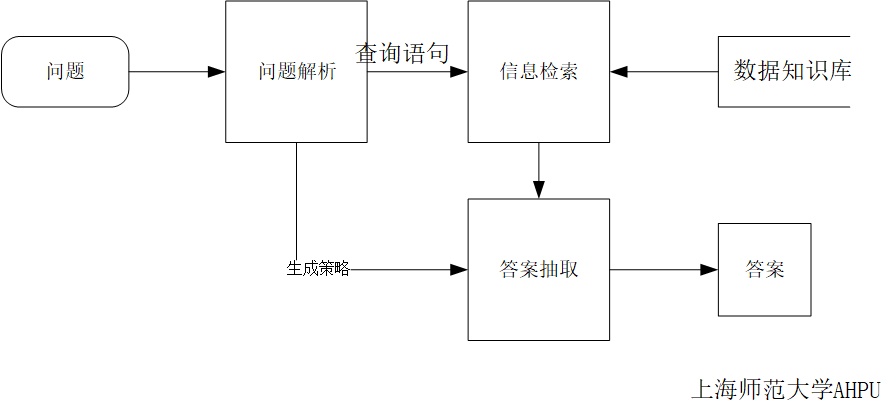1. 一种特征提取的新方法，此方法依赖于句法分析结果，通过把主干词和疑问词及其附属成分作为BN 的特征输入。
2. 用HowNet 语义词典，把疑问句、句法结构、疑问意向词在知网中的首义原作为特征输入， 采用EM 分类器。
3. 提取问题疑问词、关键核心词的主要义原、核心关键词的首义原、问句主谓宾的主要义原、命名实体、名词单复数等六种特征，采用SVM 分类器对事实疑问句进行不同特征组合的分类对比。

1. 布尔模型是一种简单检索模型，基于集合论和布尔代数。其查询由联接符AND、OR 和NOT 构成，通过对每个关键词对应的倒排索引取交集、并集或补集，返回若干相关文档给用户

2. 向量空间模型是现在的文本检索系统以及网络搜索引擎的基础， 它把文档以及用户的查询都表示成向量空间中的点，用它们之间夹角的余弦值作为相似性度量。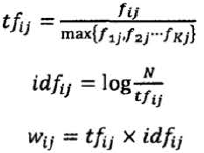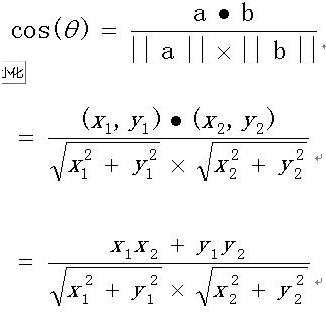3. 概率检索模型通常利用关键词作为线索，通过统计得到每个关键词在相关的文档集中出现和不出现的概率以及其在与该查询不相关的文档集中出现和不出现的概率，最终根据这些概率值，计算问句和文档的相似度。

PRP（probability ranking principle）：概率排序原理，利用概率模型来估计每篇文档和需求相关概率，然后对结果进行排序。

## d. 体系结构和各个部分细分，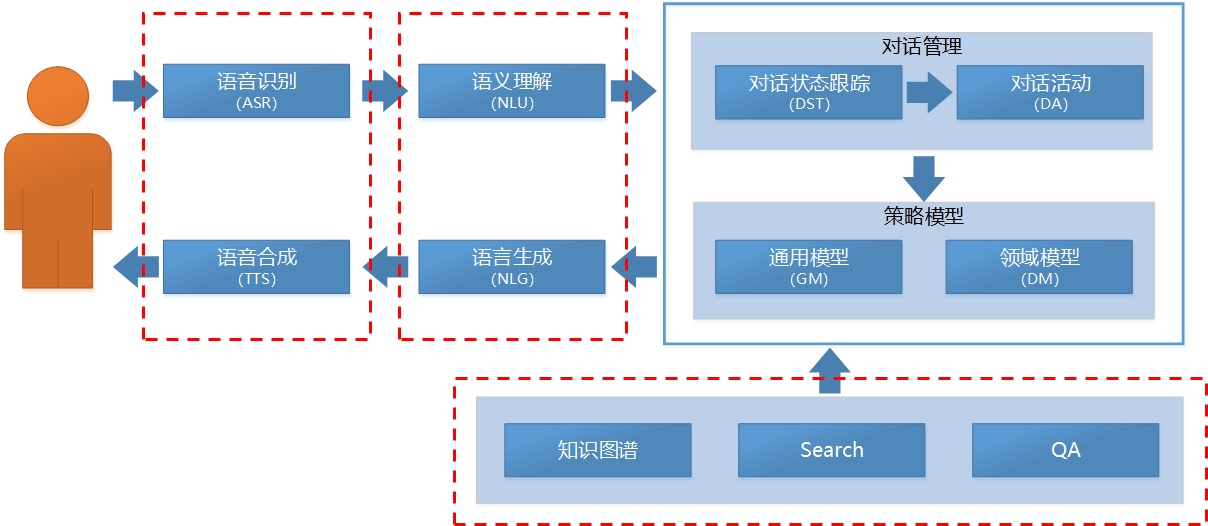#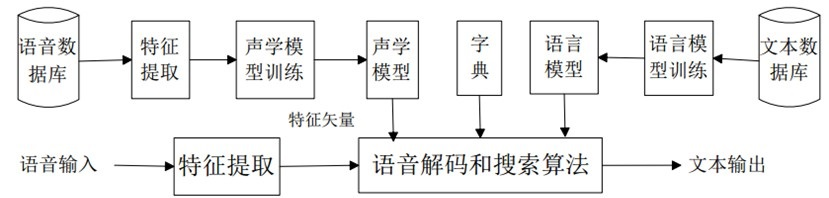1. 语音输入——这个过程可以通过电脑上的声卡来获取麦克风中输入的音频信号，或者直接读取电脑中已经存在的音频文件；
2. 音频信号特征提取——在得到音频信号之后，需要对音频信号进行预处理，然后对预处理之后的音频信号进程特征提取，MFCC是最常用的声学特征；
3. 声学模型处理——把语音的声学特征分类对应到音素或字词这样的单元；
4. 语言模型处理——用语言模型接着把字词解码成一个完整的句子，于是就得到了最终的语音识别结果。
5. 语音输出

## 各个步骤详述

1语言输入

1.1、通过电脑上的声卡读取麦克风中输入的语音信号，实现实时语音信号采集；
1.2、读取电脑本地中的.wav或.mp4文件获取语音信号。
2 音频信号特征提取
2.1 语音信号分帧处理

2.2 声学特征MFCC提取

2.3声学模型整合

2.3.1、混合声学

2.3.2、端到端的声学模型

3语音模型

## 总结：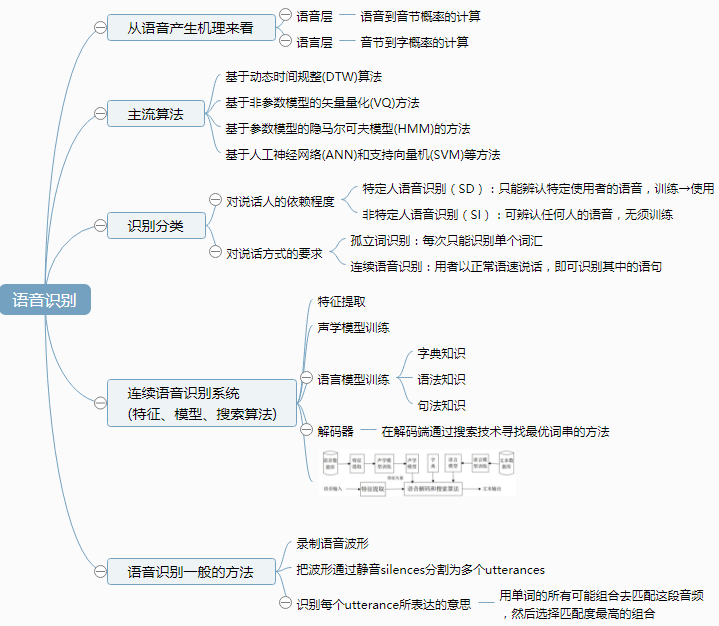1、口音和噪声

2、语义错误

3、单通道和多人会话

4、其他领域变化

5、上下文相关联判断识别

# 二.问答系统：

## 分类体系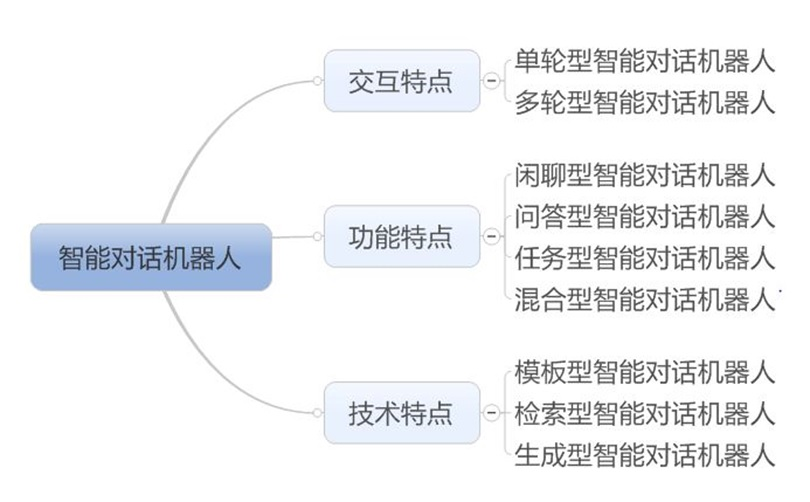## 三种形式：

1. 基于分析
2. 基于检索、
3. 基于生成。

• 基于分析的方法它是有显式的内部表达，基于检索和生成，就是部分有或者没有显性的这个内部的表示，这是这三个不同的方法的特点。
• 基于检索的方法在聊天机器人中用的多，而问答系统中也是如此，只是将对话局限在问答中。
• 基于生成的方法。在深度学习出来之前，这是有难度的，现在有深度学习基于生成对话的技术，其原理上是把问句，转化成内部表示，再把内部表示为答句。在生成器和分析器中用了大量的训练语料，基于神经网络来训练表示实现。

## 技术架构图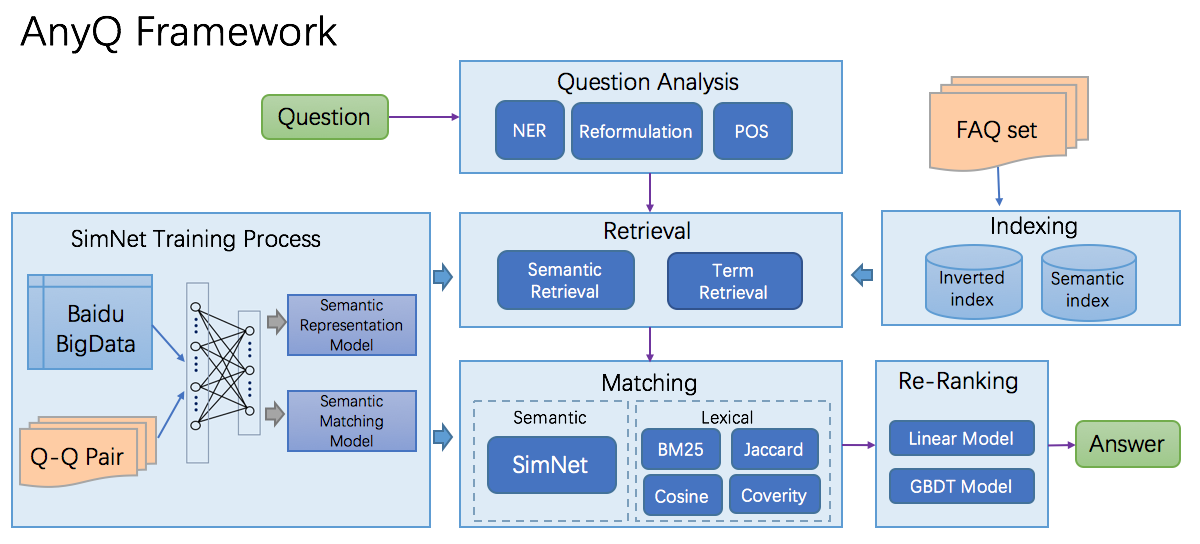1. 提问处理模块：负责对用户的提问进行处理；生成查询关键词（提问关键词，扩展关键词，...）；确定提问答案类型（PER, LOC, ORG, TIM, NUM, ...）以及提问的句法、语义表示等等。
2. 检索模块：根据提问处理模块生成的查询关键词，使用传统检索方式，检索出和提问相关的信息；返回的信息可以是段落、也可以是句群或者句子。
3. 答案抽取模块：从检索模块检索出的相关段落、或句群、或句子中抽取出和提问答案类型一致的实体，根据某种原则对候选答案进行打分，把概率最大的候选答案返回给用户

## 模型逻辑图如下：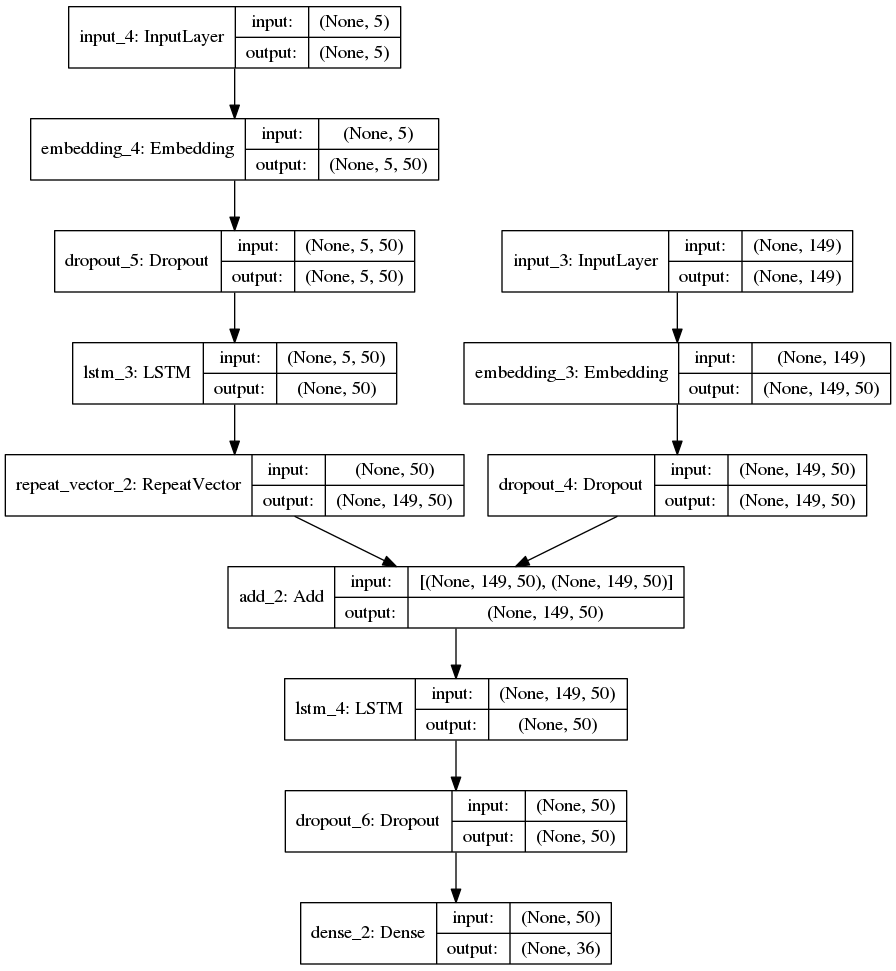# 三. 语音合成：

## 基本原理### 2.参数合成法

参数语音合成方法的优点是其音库一般较小，并且整个系统能适应的韵律特征的范围较宽，这类合成器比特率低，音质适中；缺点是参数合成技术的算法复杂，参数多，并且在压缩比较大时，信息丢失亦大，合成出的语音总是不够自然、清晰。为了改善音质，近几年发展了混合编码技术，主要是为了改善激励信号的质量，这样，虽然比特率有所增大，但音质得到了提高。

### 3.规则合成法

这是一种高级的合成方法。规则合成方法通过语音学规则产生语音。合成的词汇表不是事先确定，系统中存储的是最小的语音单位的声学参数，以及由音素组成音节、由音节组成词、由词组成句子和控制音调、轻重音等韵律的各种规则。给出待合成的文本数据后，合成系统利用规则自动地将他们转换成连续的语音声波。这种方法可以合成无限词汇的语句。这种算法中，用于波形拼接和韵律控制的、较有代表性的算法是基音同步叠加技术（PSOLA），该方法既能保持所发音的主要音段特征，又能在拼接时灵活调整其基频、时长和强度等超音段特征。其核心思想是，直接对存储于音库的语音运用PSOLA算法来进行拼接，从而整合成完整的语音。有别于传统概念上只是将不同的语音单元进行简单拼接的波形编辑合成，规则合成系统首先要在大量语音库中，选择最合适的语音单元来用于拼接，并在选音过程中往往采用多种复杂的技术，最后在拼接时，要使用如PSOLA算法等，对其合成语音的韵律特征进行修改，从而使合成的语音能达到很高的音质。

## 总的流程